# Diamonds are Forever

Feature Engineering with the Diamonds Dataset
tidymodels
Author

Mark Rieke

Published

November 14, 2021

Are y’all ready for some charts?? This week, I did a bit of machine learning practice with the `diamonds dataset`. This dataset is interesting and good for practice for a few reasons:

• there are lots of observations (50,000+);
• it includes a mix of numeric and categorical variables;
• there are some data oddities to deal with (log scales, interactions, non-linear relations)

I’ll be doing a bit of feature engineering prior to fitting an tuning a linear model that predicts the each diamond’s `price` with the `glmnet` package. This will give a good end-to-end glimpse into the data exploration and model fitting process! Before we get into that, let’s load some packages and get a preview of the dataset.

Code
``````library(tidyverse)
library(tidymodels)
library(vip)

theme_set(theme_minimal())

diamonds %>%
``````#> # A tibble: 10 × 10
#>    carat cut       color clarity depth table price     x     y     z
#>    <dbl> <ord>     <ord> <ord>   <dbl> <dbl> <int> <dbl> <dbl> <dbl>
#>  1  0.23 Ideal     E     SI2      61.5    55   326  3.95  3.98  2.43
#>  2  0.21 Premium   E     SI1      59.8    61   326  3.89  3.84  2.31
#>  3  0.23 Good      E     VS1      56.9    65   327  4.05  4.07  2.31
#>  4  0.29 Premium   I     VS2      62.4    58   334  4.2   4.23  2.63
#>  5  0.31 Good      J     SI2      63.3    58   335  4.34  4.35  2.75
#>  6  0.24 Very Good J     VVS2     62.8    57   336  3.94  3.96  2.48
#>  7  0.24 Very Good I     VVS1     62.3    57   336  3.95  3.98  2.47
#>  8  0.26 Very Good H     SI1      61.9    55   337  4.07  4.11  2.53
#>  9  0.22 Fair      E     VS2      65.1    61   337  3.87  3.78  2.49
#> 10  0.23 Very Good H     VS1      59.4    61   338  4     4.05  2.39``````

Since we’re predicting price, let’s look at its distribution first.

Code
``````diamonds %>%
ggplot(aes(x = price)) +
geom_histogram()``````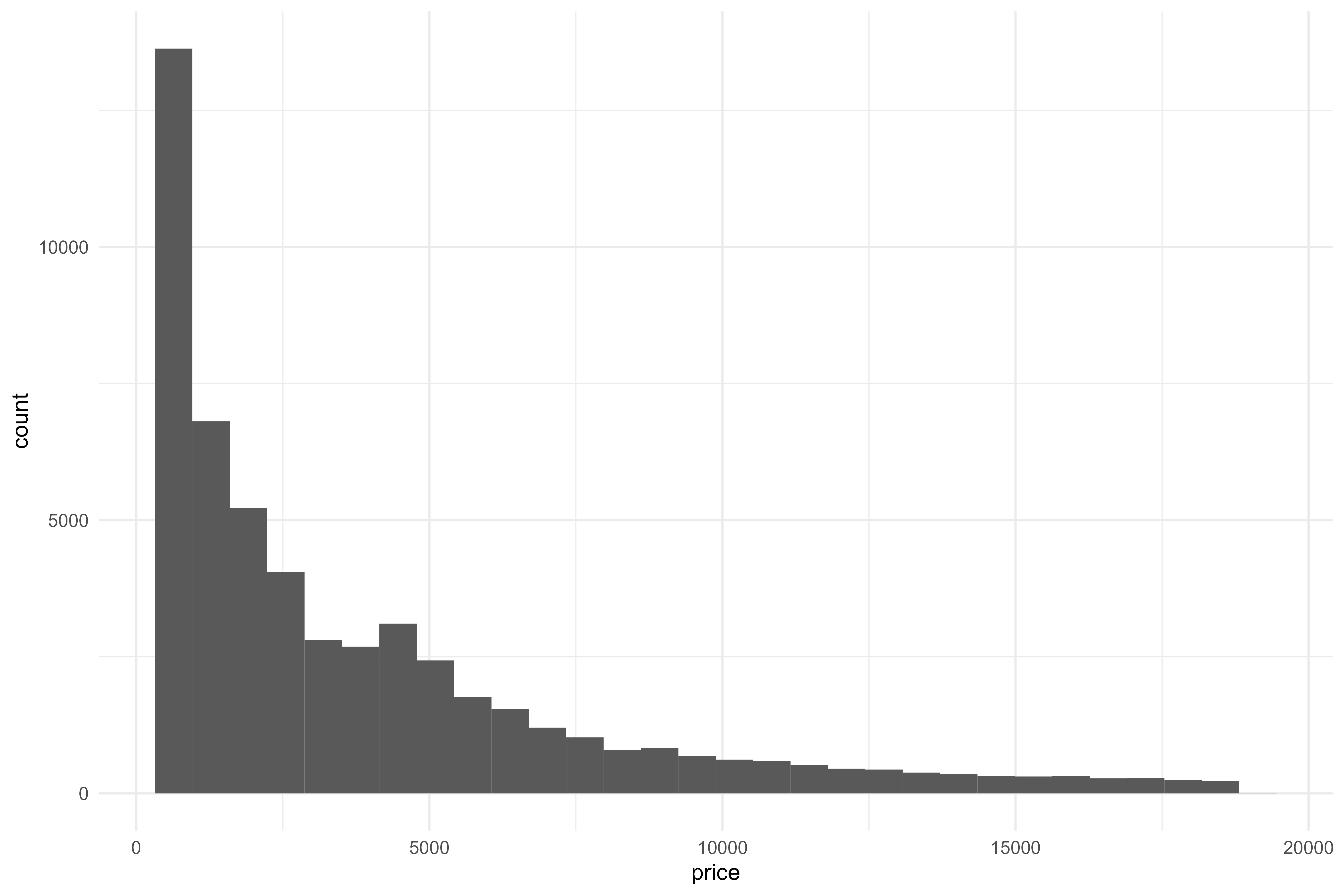We’re definitely gonna want to apply a transformation to the price when modeling - let’s look at the distribution on a log-10 scale.

Code
``````diamonds %>%
ggplot(aes(x = price)) +
geom_histogram() +
scale_x_log10()``````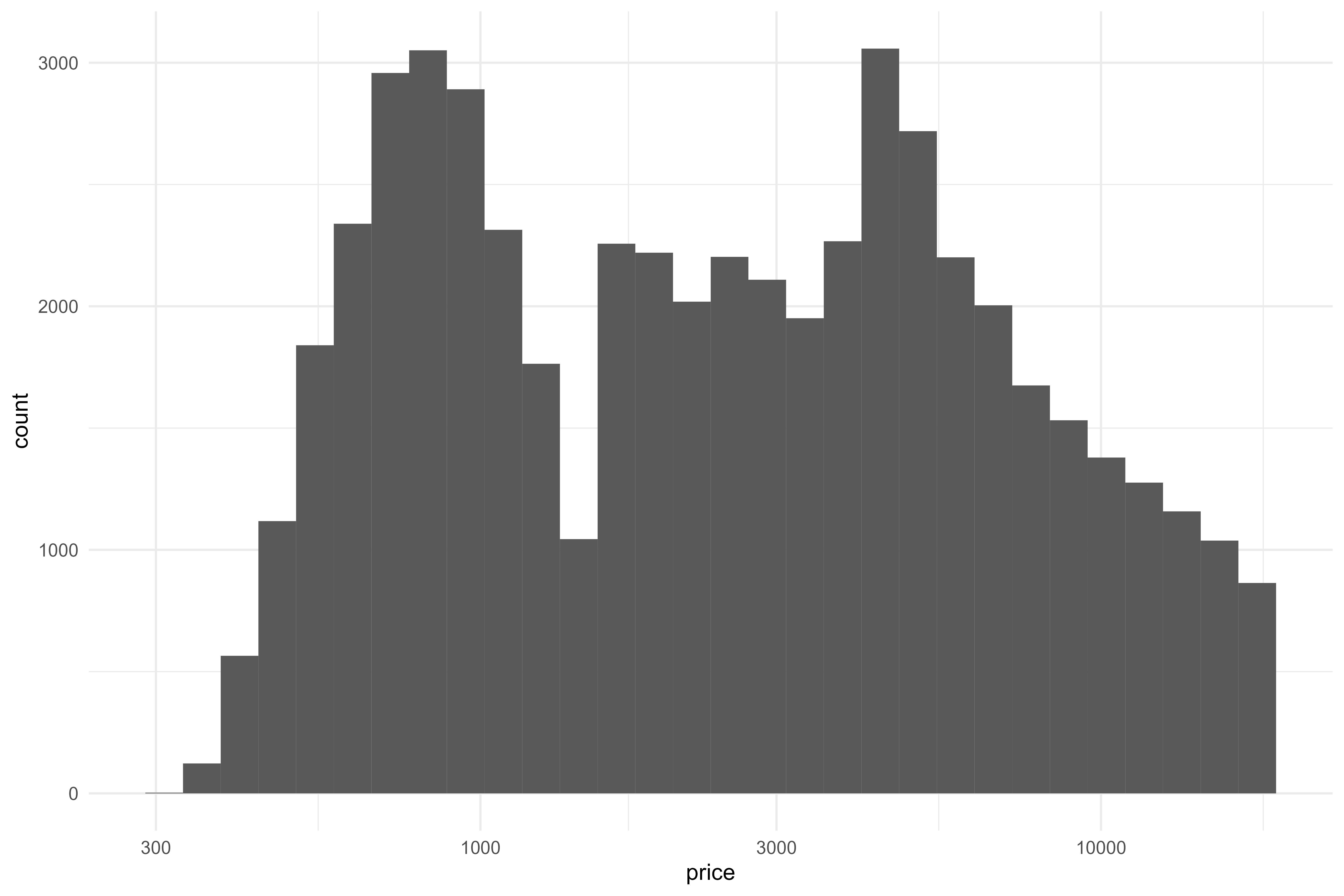That’s a lot more evenly distributed, if not perfect. That’s a fine starting point, so now we’ll look through the rest of the data.

Code
``````diamonds %>%
ggplot(aes(x = carat)) +
geom_histogram()``````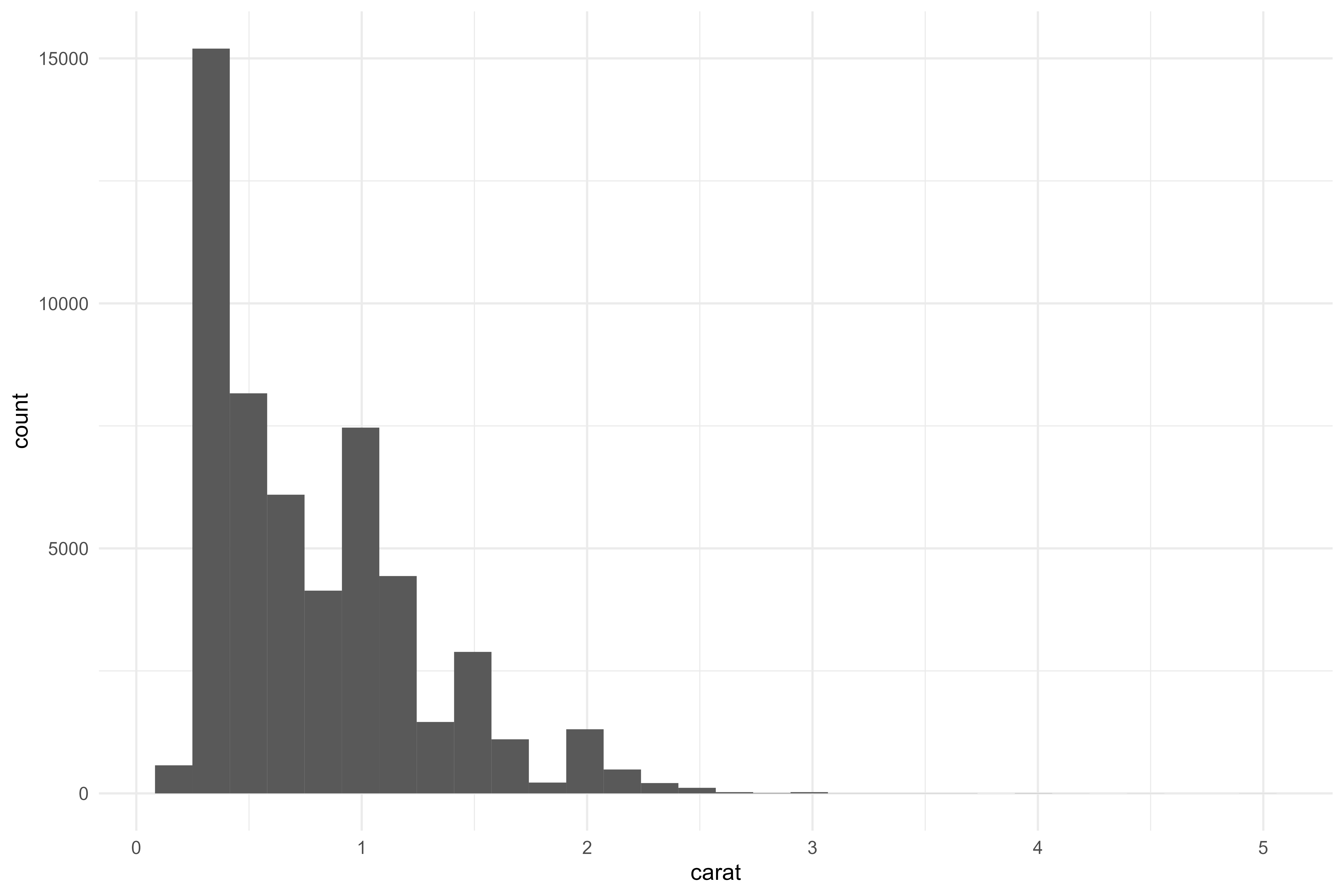Code
``````diamonds %>%
ggplot(aes(x = cut,
y = price)) +
geom_boxplot()``````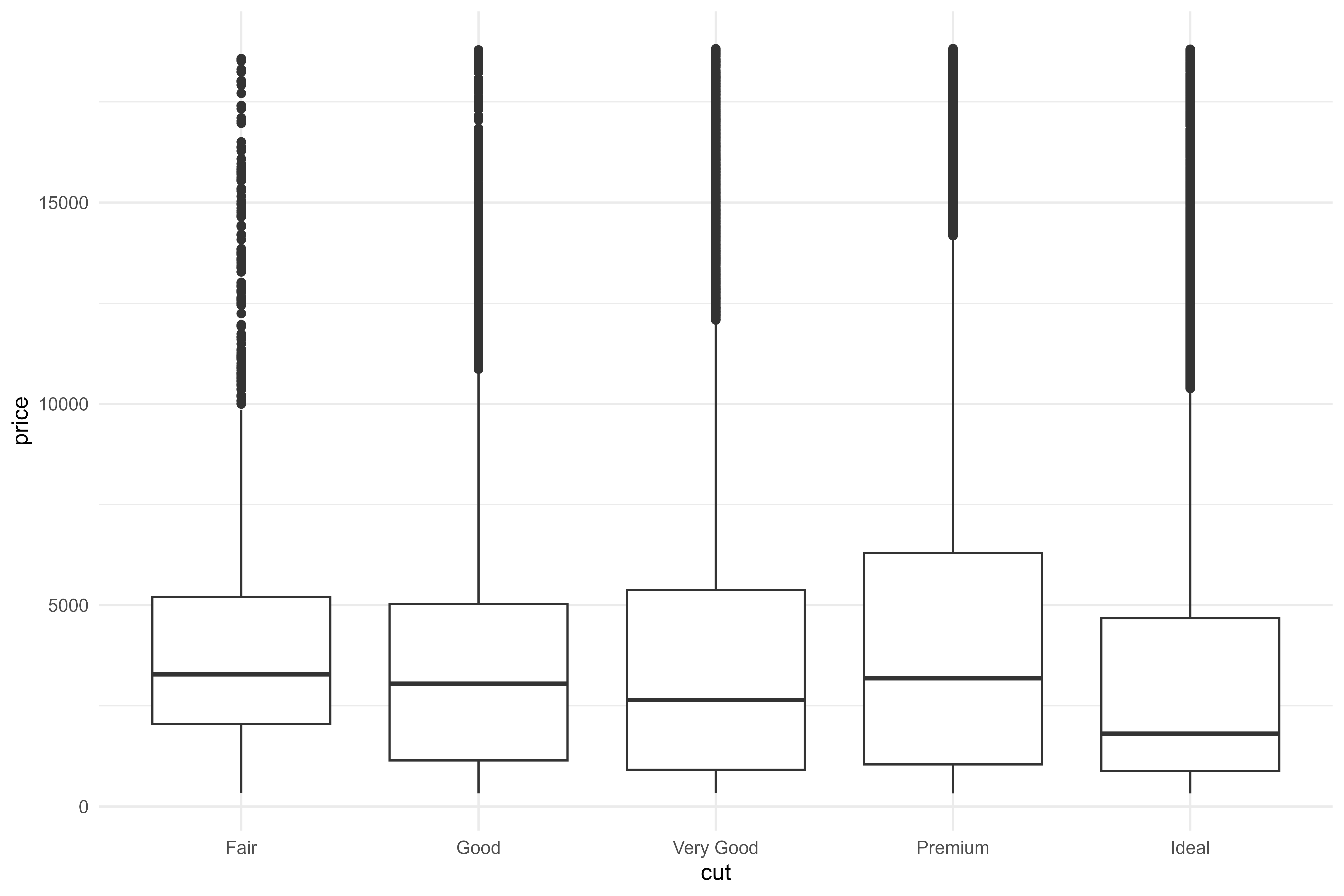Code
``````diamonds %>%
count(cut) %>%
ggplot(aes(x = cut,
y = n)) +
geom_col()``````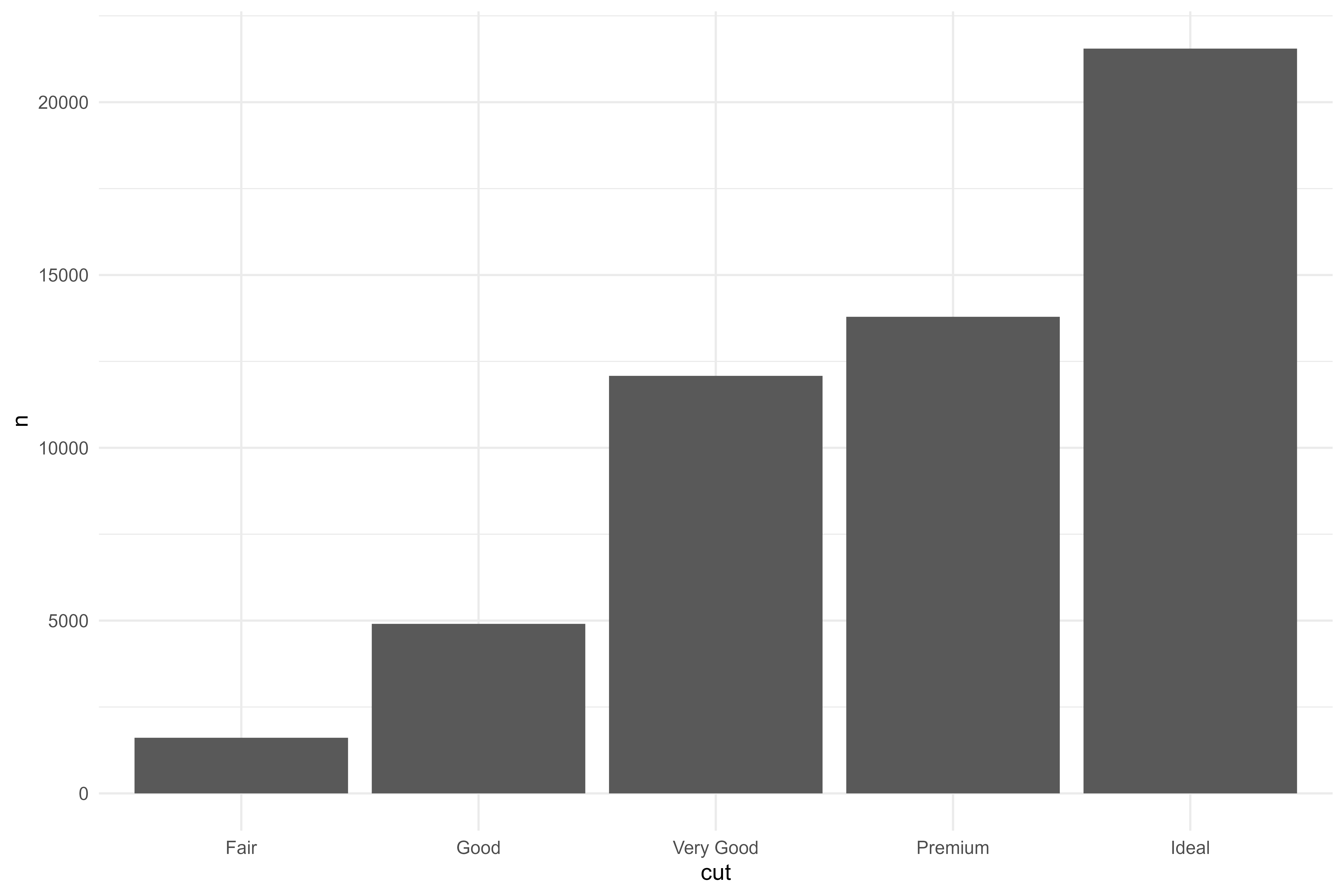Code
``````diamonds %>%
ggplot(aes(x = color,
y = price)) +
geom_boxplot()``````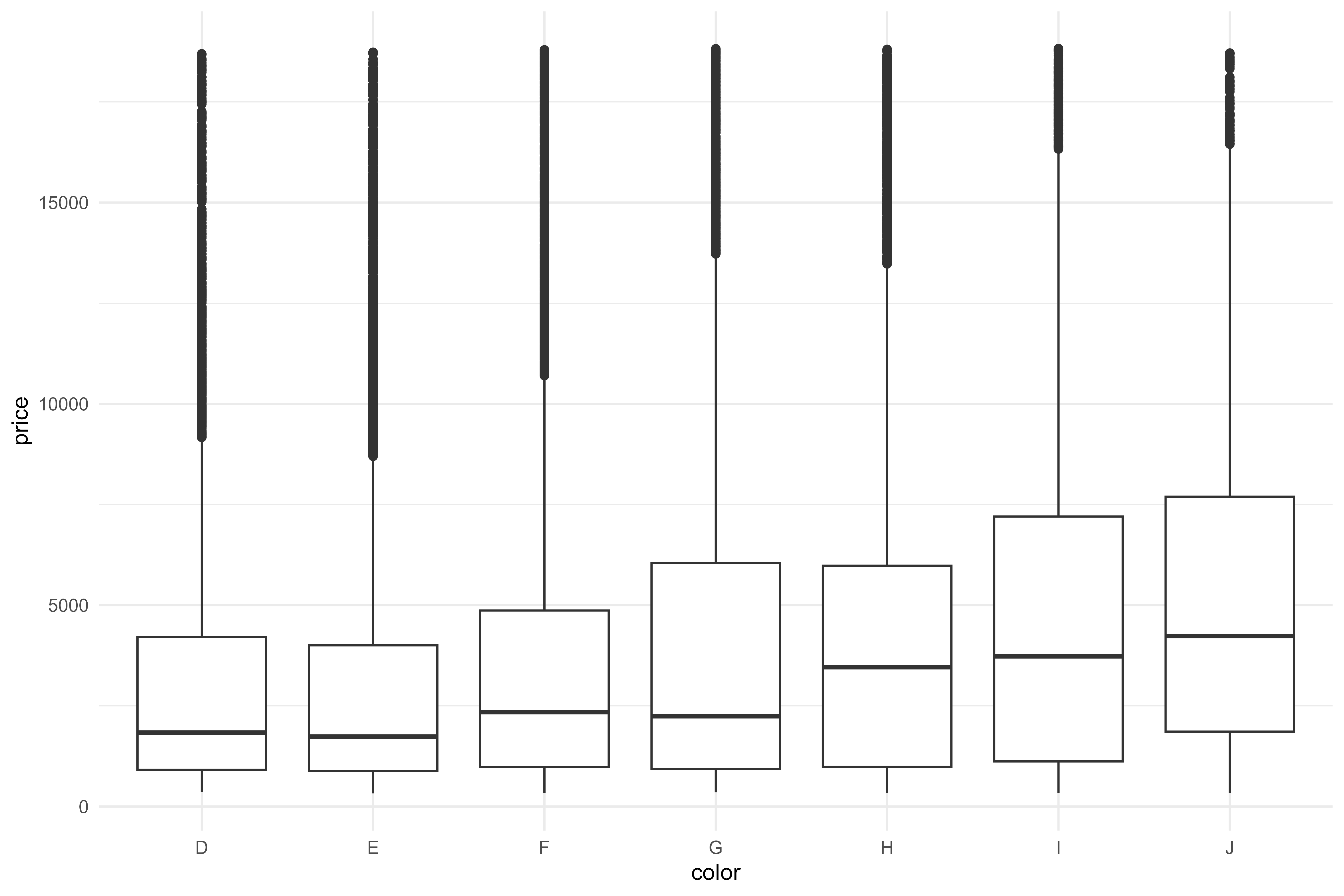Code
``````diamonds %>%
count(color) %>%
ggplot(aes(x = color,
y = n)) +
geom_col()``````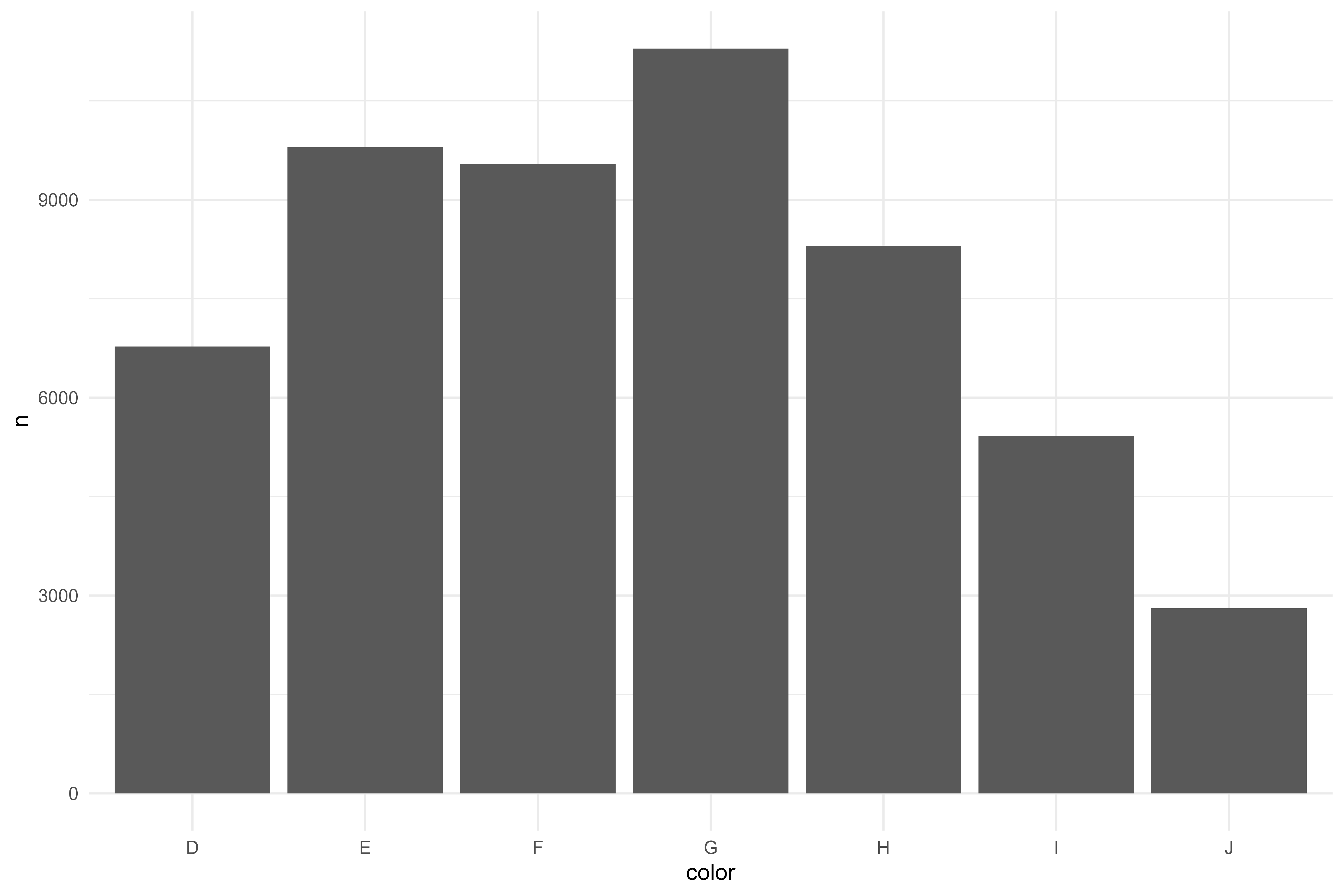Code
``````diamonds %>%
ggplot(aes(x = clarity,
y = price)) +
geom_boxplot()``````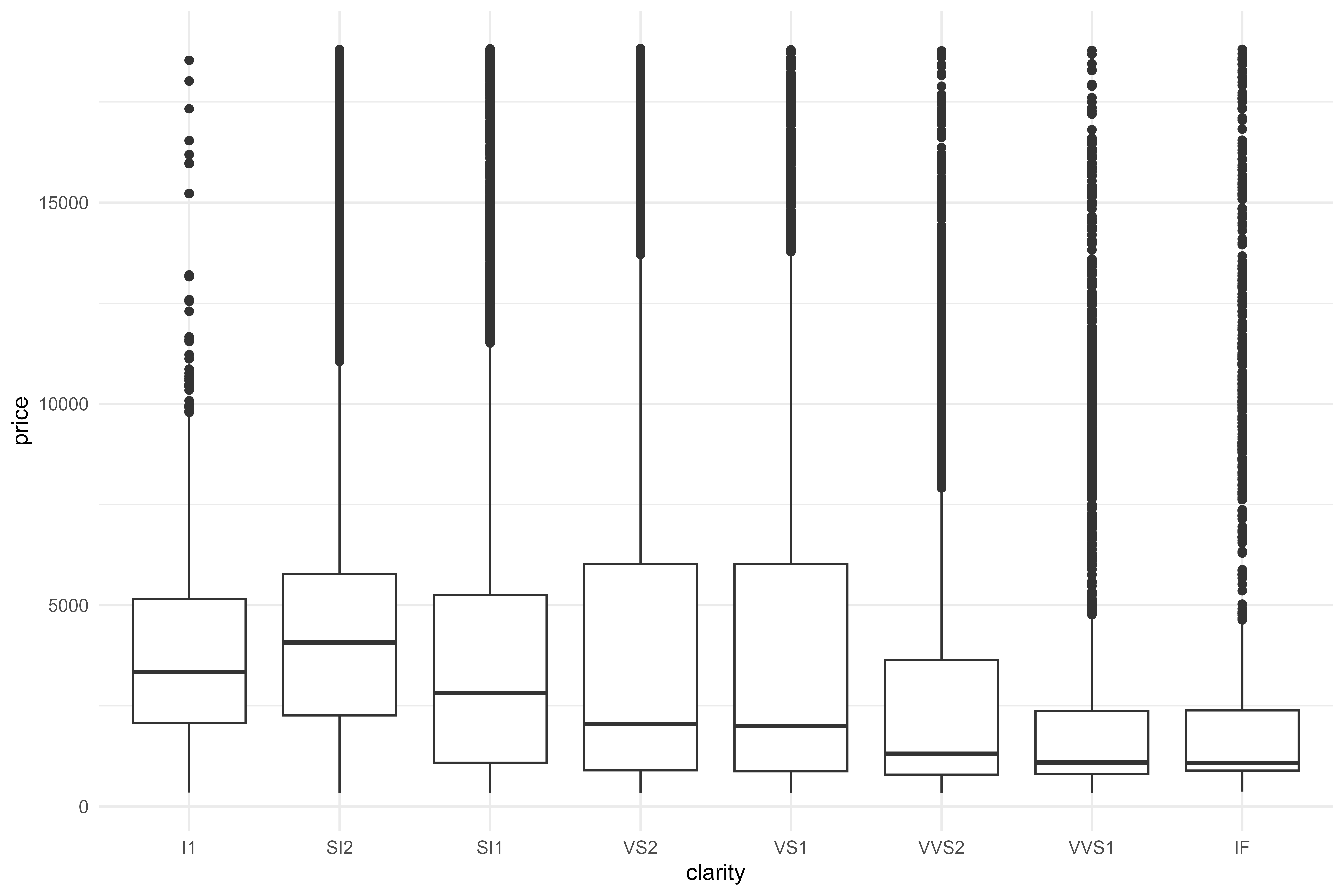Code
``````diamonds %>%
count(clarity) %>%
ggplot(aes(x = clarity,
y = n)) +
geom_col()``````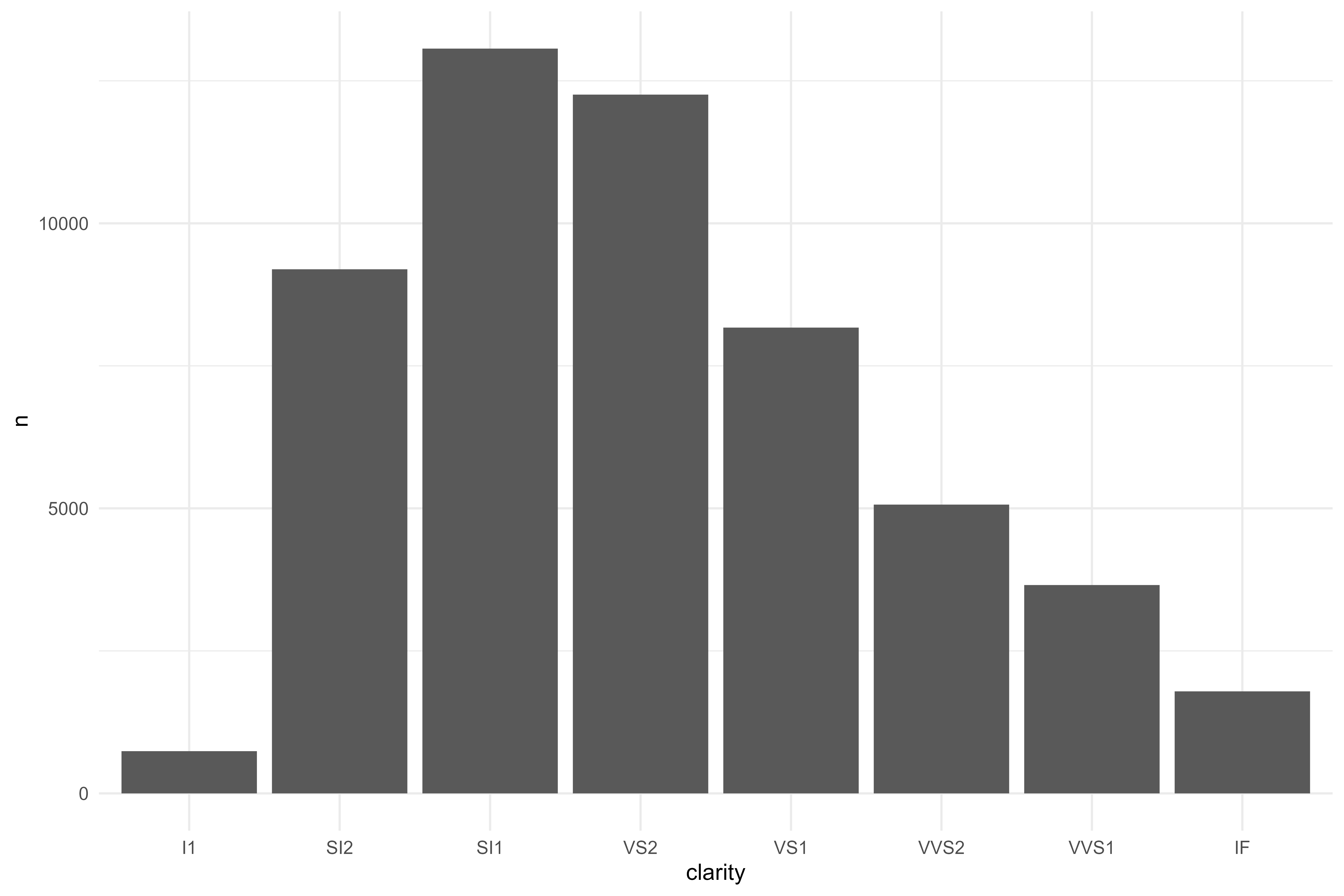Code
``````diamonds %>%
ggplot(aes(x = depth)) +
geom_histogram()``````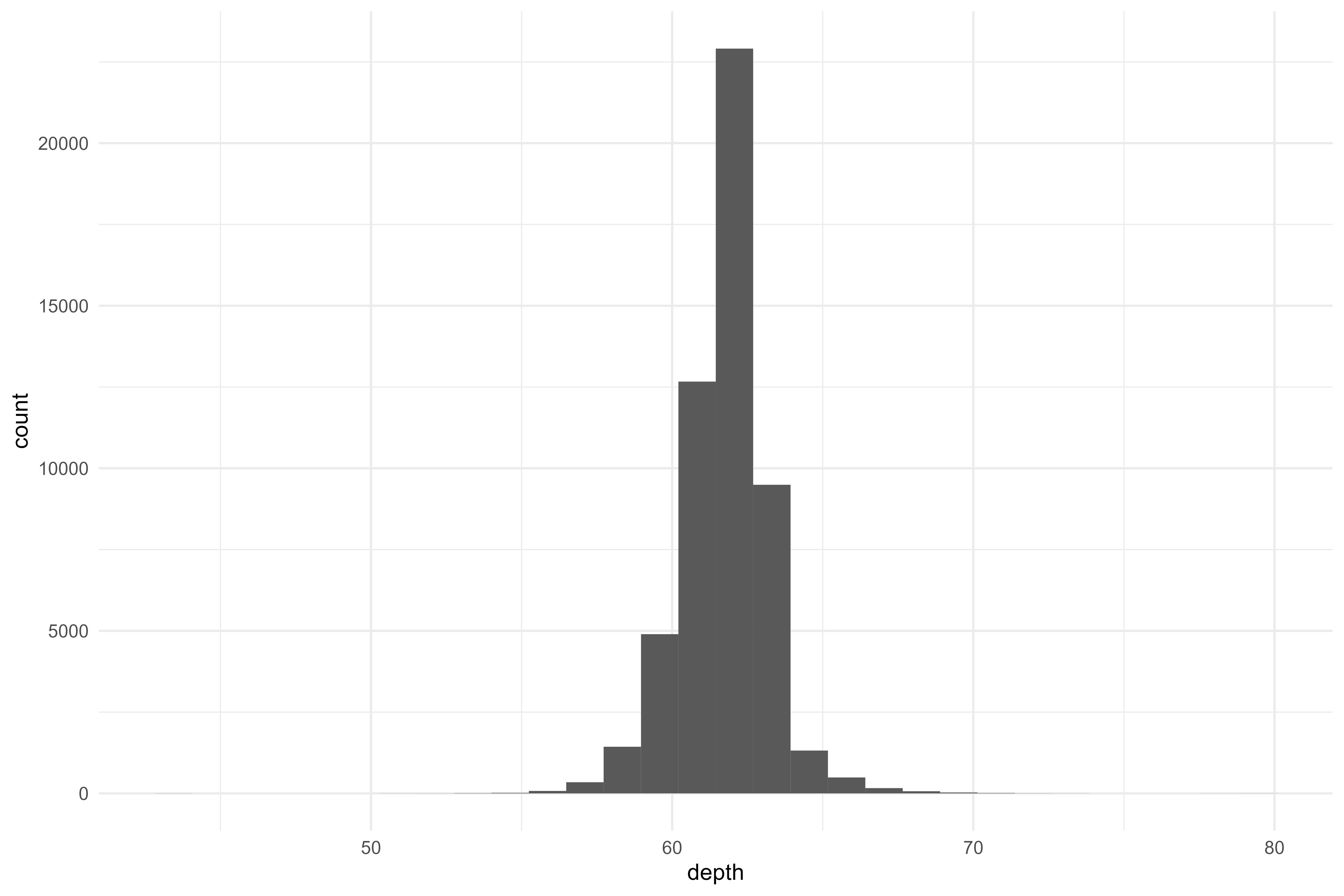Code
``````diamonds %>%
ggplot(aes(x = table)) +
geom_histogram() ``````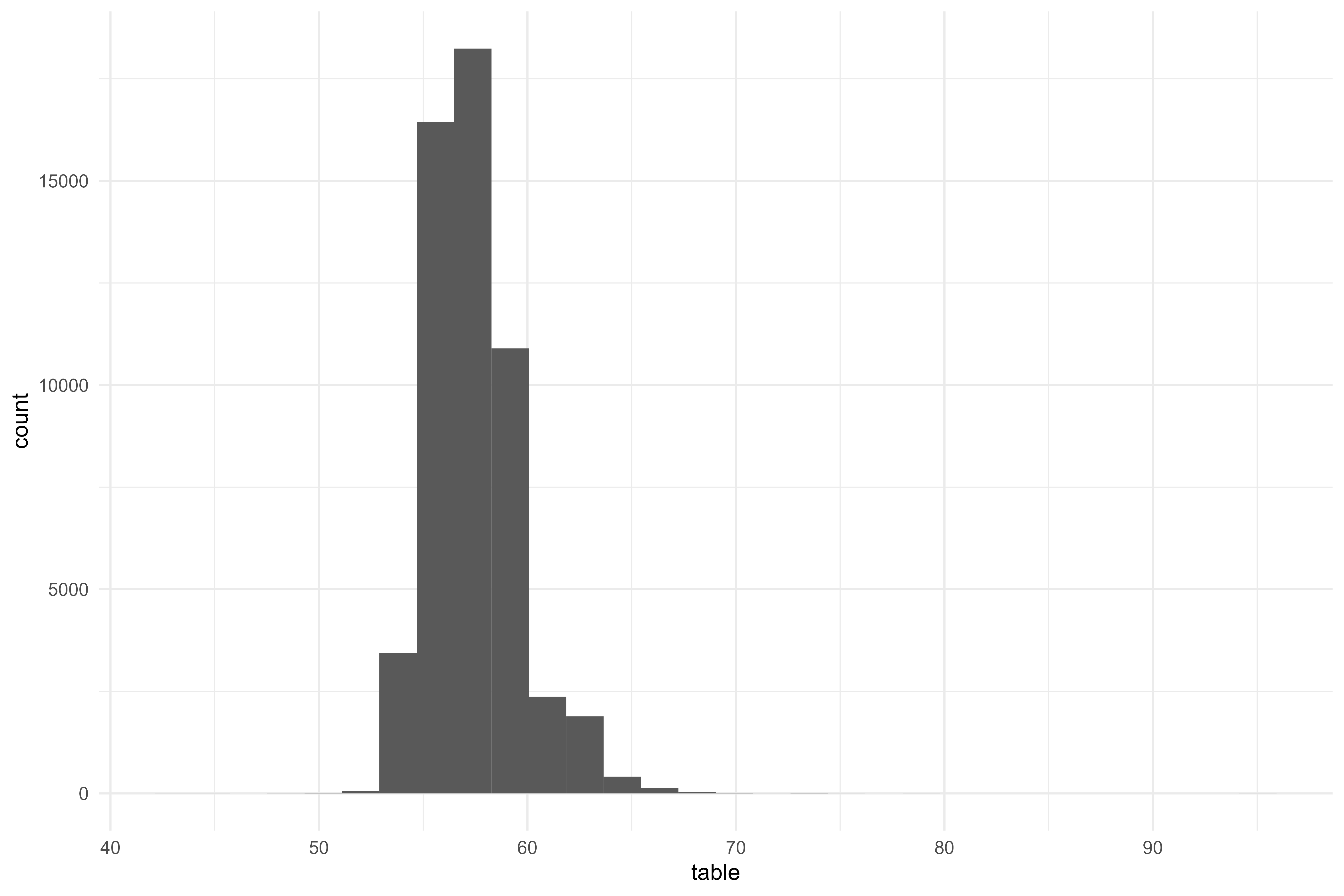Code
``````diamonds %>%
ggplot(aes(x = x)) +
geom_histogram()``````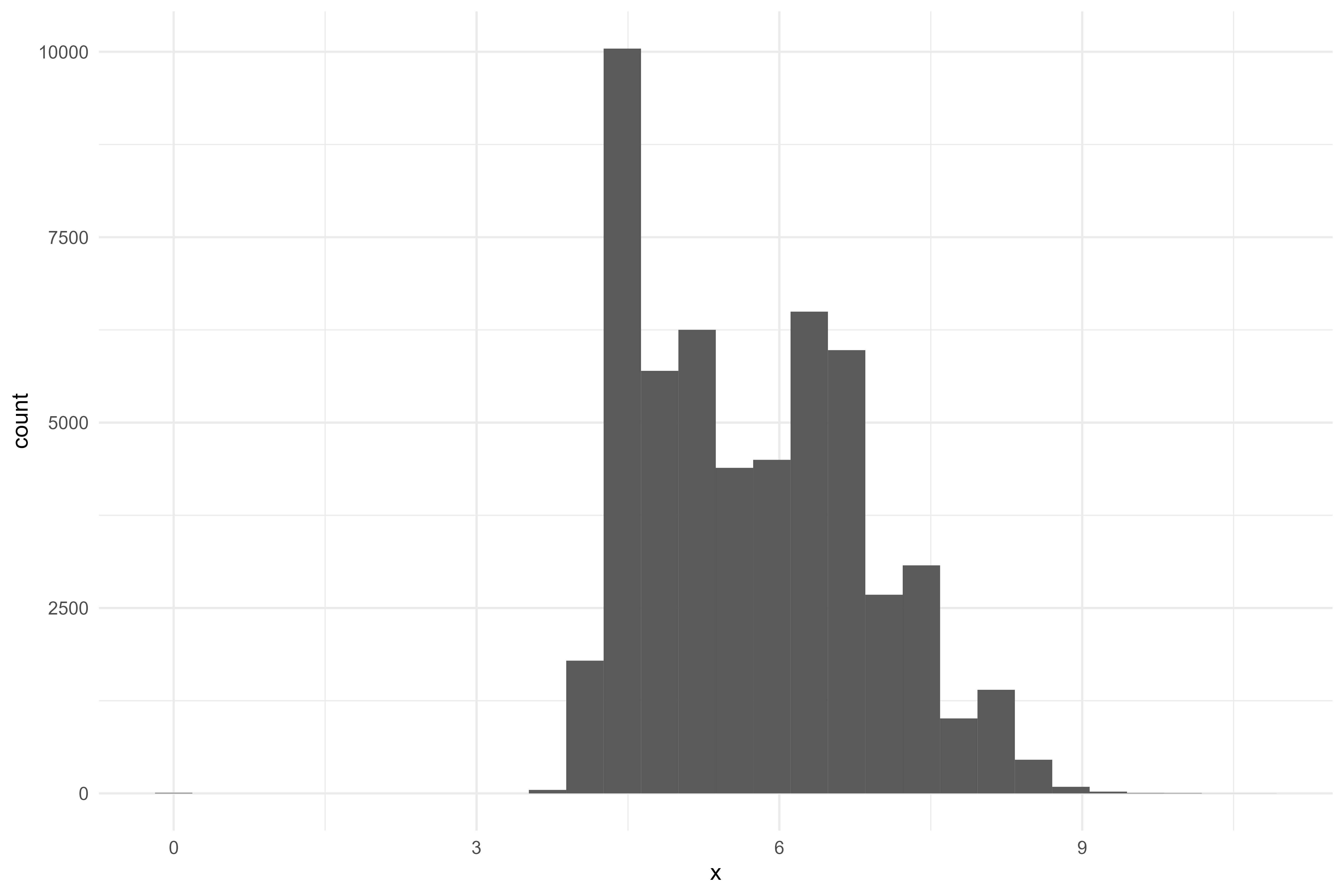Code
``````diamonds %>%
ggplot(aes(x = y)) +
geom_histogram() ``````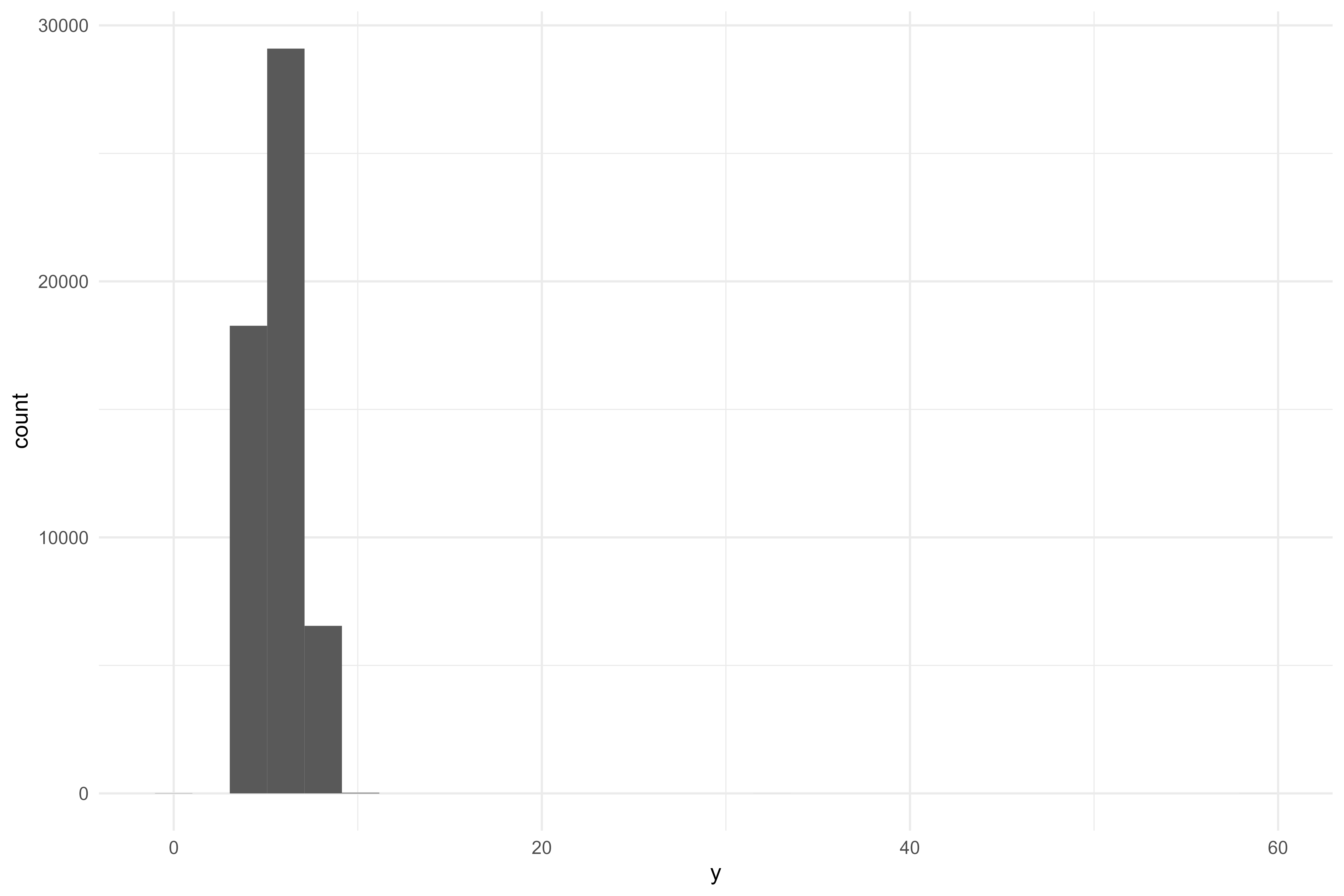Code
``````diamonds %>%
ggplot(aes(x = z)) +
geom_histogram()``````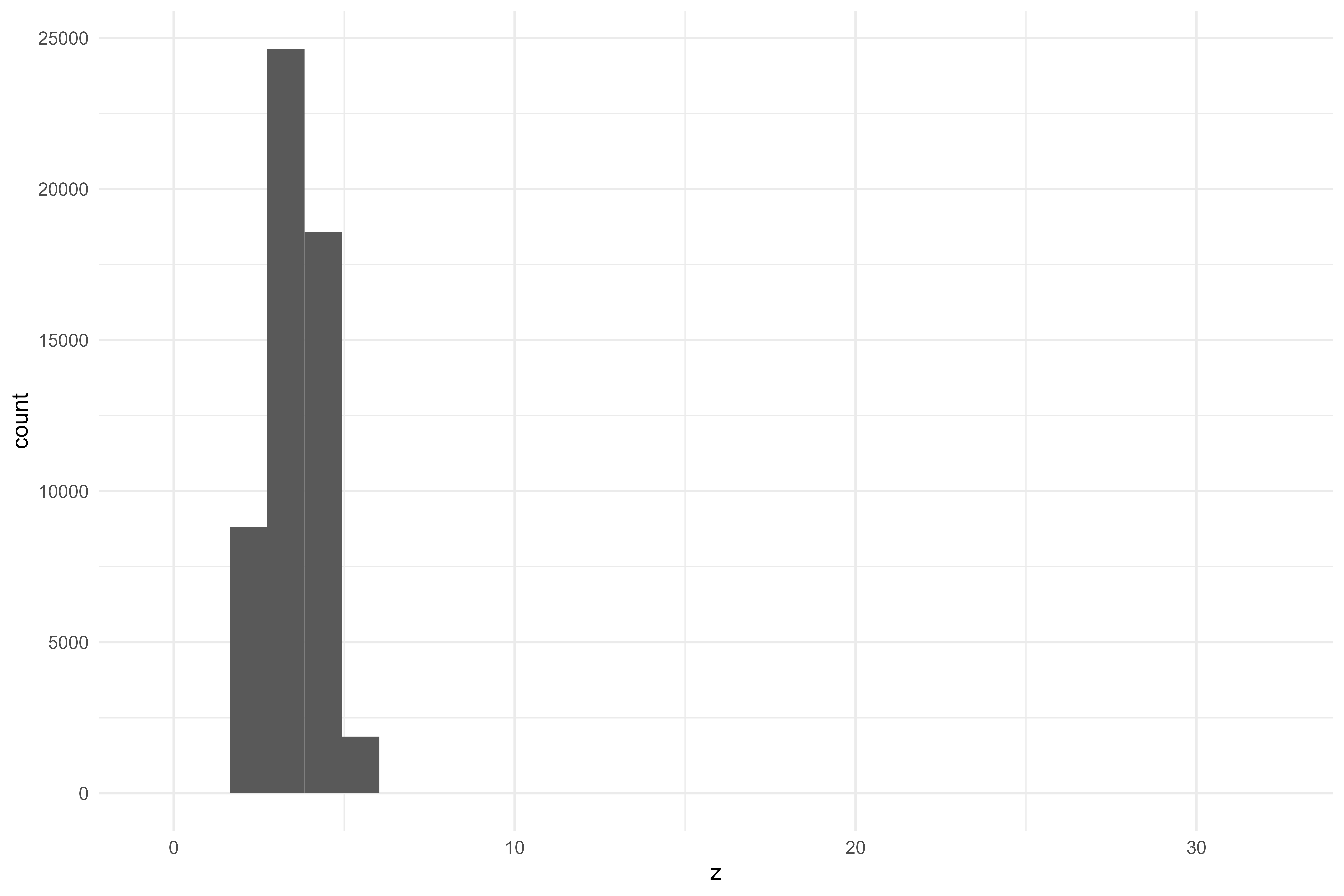It looks like there may be a good opportunity to try out a few normalization and resampling techniques, but before we get into any of that, let’s build a baseline linear model.

Code
``````# splits
diamonds_split <- initial_split(diamonds)
diamonds_train <- training(diamonds_split)
diamonds_test <- testing(diamonds_split)

# resamples (don't want to use testing data!)
diamonds_folds <- vfold_cv(diamonds_train)

# model spec
mod01 <-
linear_reg() %>%
set_engine("lm")

# recipe
rec01 <-
recipe(price ~ ., data = diamonds_train) %>%
step_dummy(all_nominal_predictors())

# controls
ctrl_preds <-
control_resamples(save_pred = TRUE)

# create a wf
wf01 <-
workflow() %>%

# parallel processing
doParallel::registerDoParallel()

# fit
rs01 <-
fit_resamples(
wf01,
diamonds_folds,
control = ctrl_preds
)

# metrics!
collect_metrics(rs01)``````
``````#> # A tibble: 2 × 6
#>   .metric .estimator     mean     n  std_err .config
#>   <chr>   <chr>         <dbl> <int>    <dbl> <chr>
#> 1 rmse    standard   1127.       10 8.71     Preprocessor1_Model1
#> 2 rsq     standard      0.920    10 0.000912 Preprocessor1_Model1``````

And right off the bat, we can see a fairly high value for `rsq`! However, `rsq` doesn’t tell the whole story, so we should check our predictions and residuals plots.

Code
``````augment(rs01) %>%
ggplot(aes(x = price,
y = .pred)) +
geom_point(alpha = 0.01) +
geom_abline(linetype = "dashed",
size = 0.1,
alpha = 0.5)``````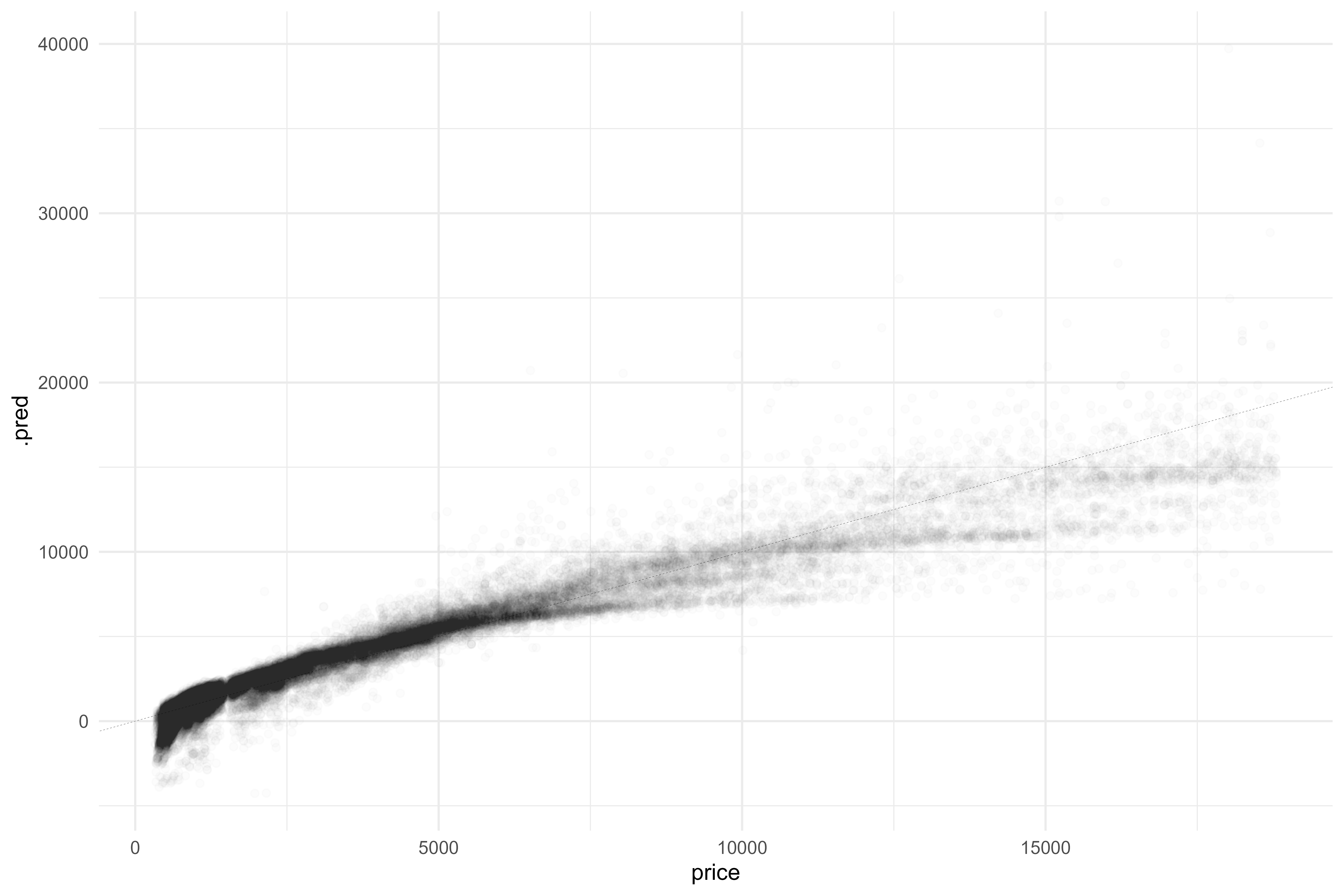This is definitely not what we want to see! It looks like there’s an odd curve/structure to the graph and we’re actually predicting quite a few negative values. The residuals plot doesn’t look too great either.

Code
``````augment(rs01) %>%
ggplot(aes(x = price,
y = .resid)) +
geom_point(alpha = 0.01) +
geom_hline(yintercept = 0,
linetype = "dashed",
alpha = 0.5,
size = 0.1)``````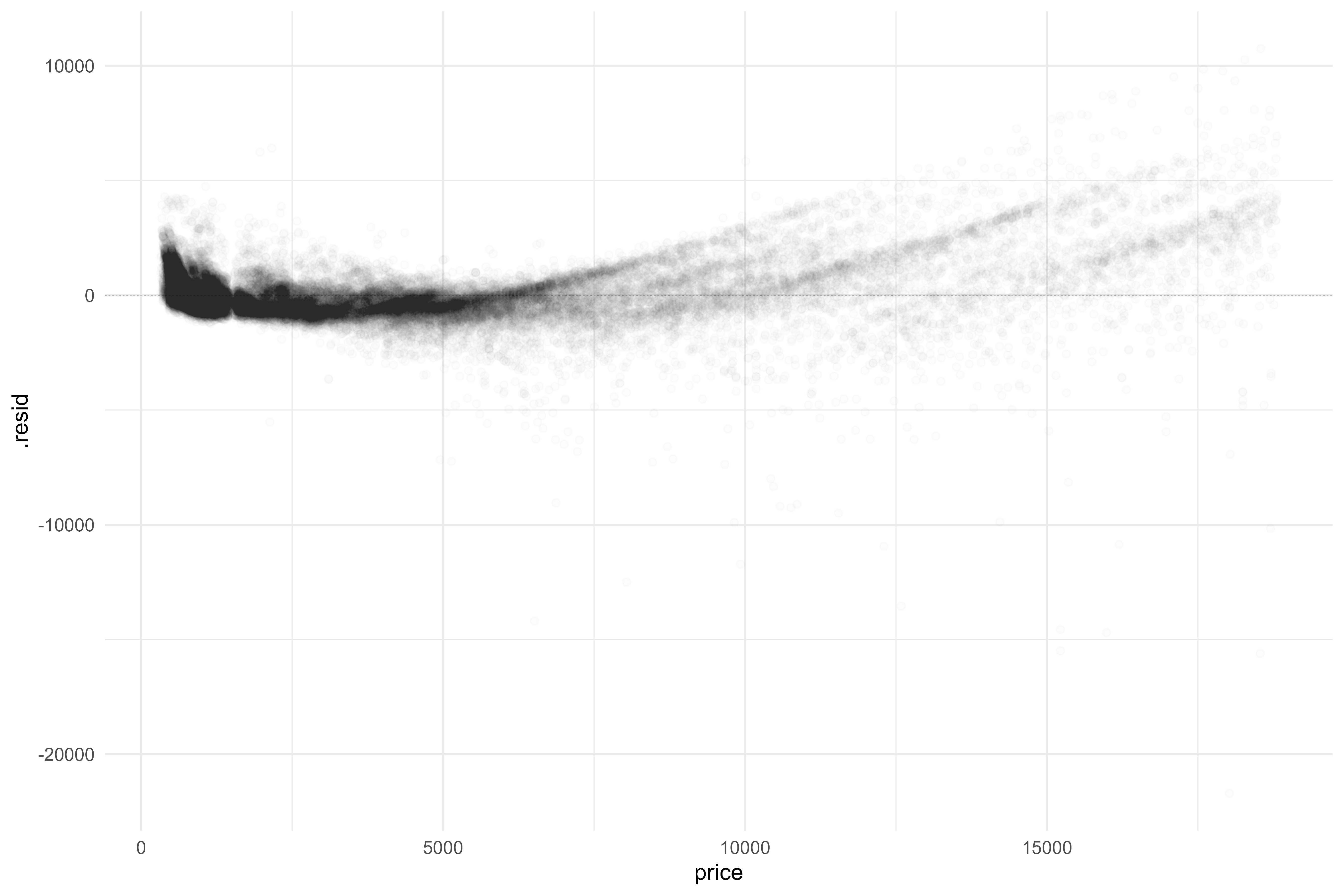What we’d like to see is a 0-correlation plot with errors normally distributed; what we’re seeing instead, however, is a ton of structure.

That being said, that’s okay! we expected this first pass to be pretty rough! And the price is clearly on a log-10 scale. To make apples-apples comparisons with models going forward, I’ll retrain this basic linear model to predict the `log10(price)`. This’ll involve a bit of data re-manipulation!

Code
``````# log transform price
diamonds_model <-
diamonds %>%
mutate(price = log10(price),
across(cut:clarity, as.character))

# bad practice copy + paste lol

# splits
set.seed(999)
diamonds_split <- initial_split(diamonds_model)
diamonds_train <- training(diamonds_split)
diamonds_test <- testing(diamonds_split)

# resamples (don't want to use testing data!)
set.seed(888)
diamonds_folds <- vfold_cv(diamonds_train)

# model spec
mod01 <-
linear_reg() %>%
set_engine("lm")

# recipe
rec01 <-
recipe(price ~ ., data = diamonds_train) %>%
step_dummy(all_nominal_predictors())

# controls
ctrl_preds <-
control_resamples(save_pred = TRUE)

# create a wf
wf01 <-
workflow() %>%

# parallel processing
doParallel::registerDoParallel()

# fit
set.seed(777)
rs01 <-
fit_resamples(
wf01,
diamonds_folds,
control = ctrl_preds
)

# metrics!
collect_metrics(rs01)``````
``````#> # A tibble: 2 × 6
#>   .metric .estimator   mean     n std_err .config
#>   <chr>   <chr>       <dbl> <int>   <dbl> <chr>
#> 1 rmse    standard   0.0793    10 0.00557 Preprocessor1_Model1
#> 2 rsq     standard   0.966     10 0.00494 Preprocessor1_Model1``````

And wow, that one transformation increased our `rsq` to 0.96! Again, that’s not the whole story, and we’re going to be evaluating models based on the `rmse`. Let’s look at how our prediction map has updated:

Code
``````rs01 %>%
augment() %>%
ggplot(aes(x = price,
y = .pred)) +
geom_point(alpha = 0.01) +
geom_abline(linetype = "dashed",
size = 0.1,
alpha = 0.5) ``````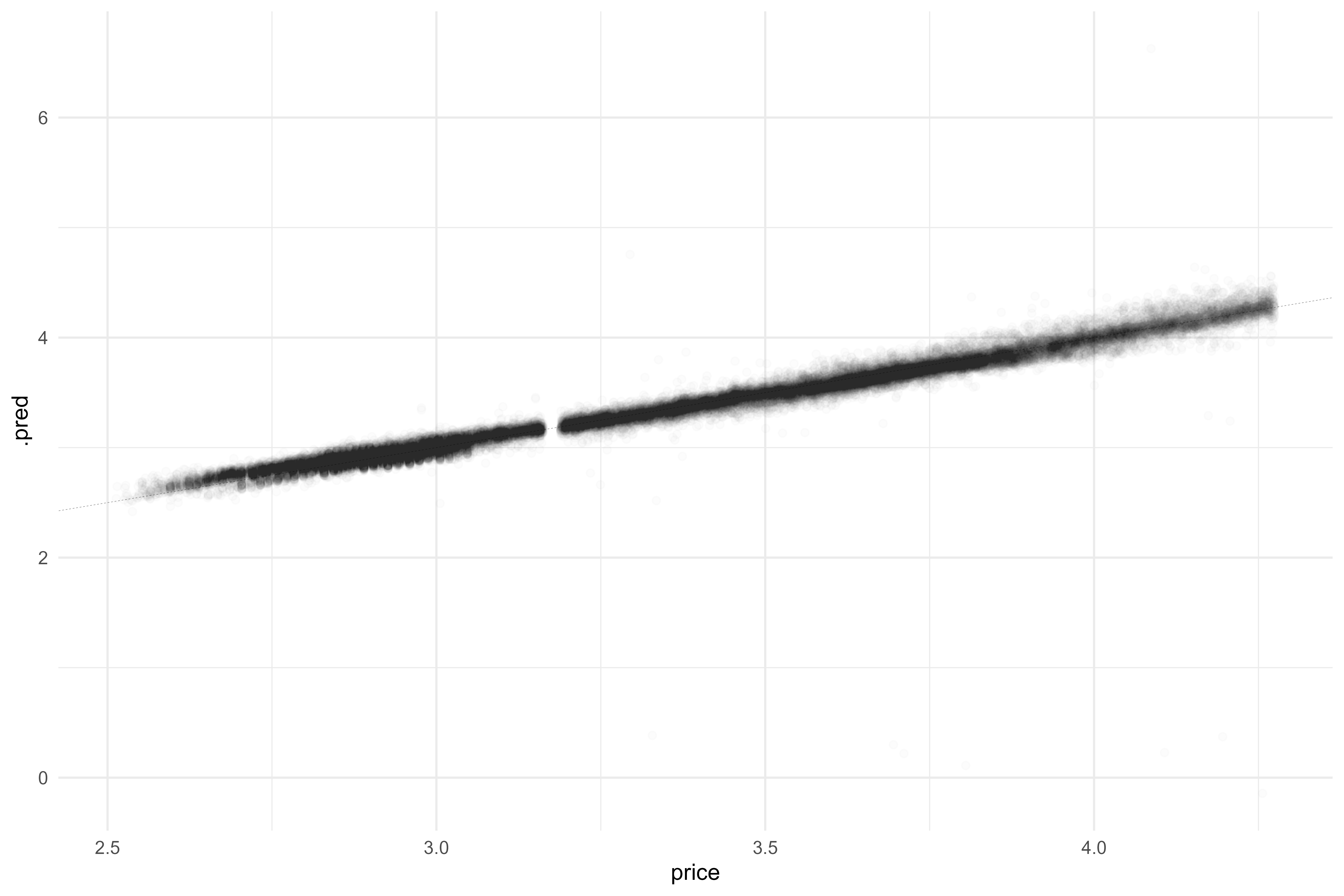Now that is a much better starting place to be at! Let’s look at our coefficients

Code
``````set.seed(666) # :thedevilisalive:
wf01 %>%
fit(diamonds_train) %>%
pull_workflow_fit() %>%
vip::vi() %>%
mutate(Variable = fct_reorder(Variable, Importance)) %>%
ggplot(aes(x = Variable,
y = Importance,
fill = Sign)) +
geom_col() +
coord_flip() +
theme(plot.title.position = "plot") +
labs(x = NULL,
y = NULL,
title = "Diamonds are forever",
subtitle = "Variable importance plot of a basic linear regression predicting diamond price")``````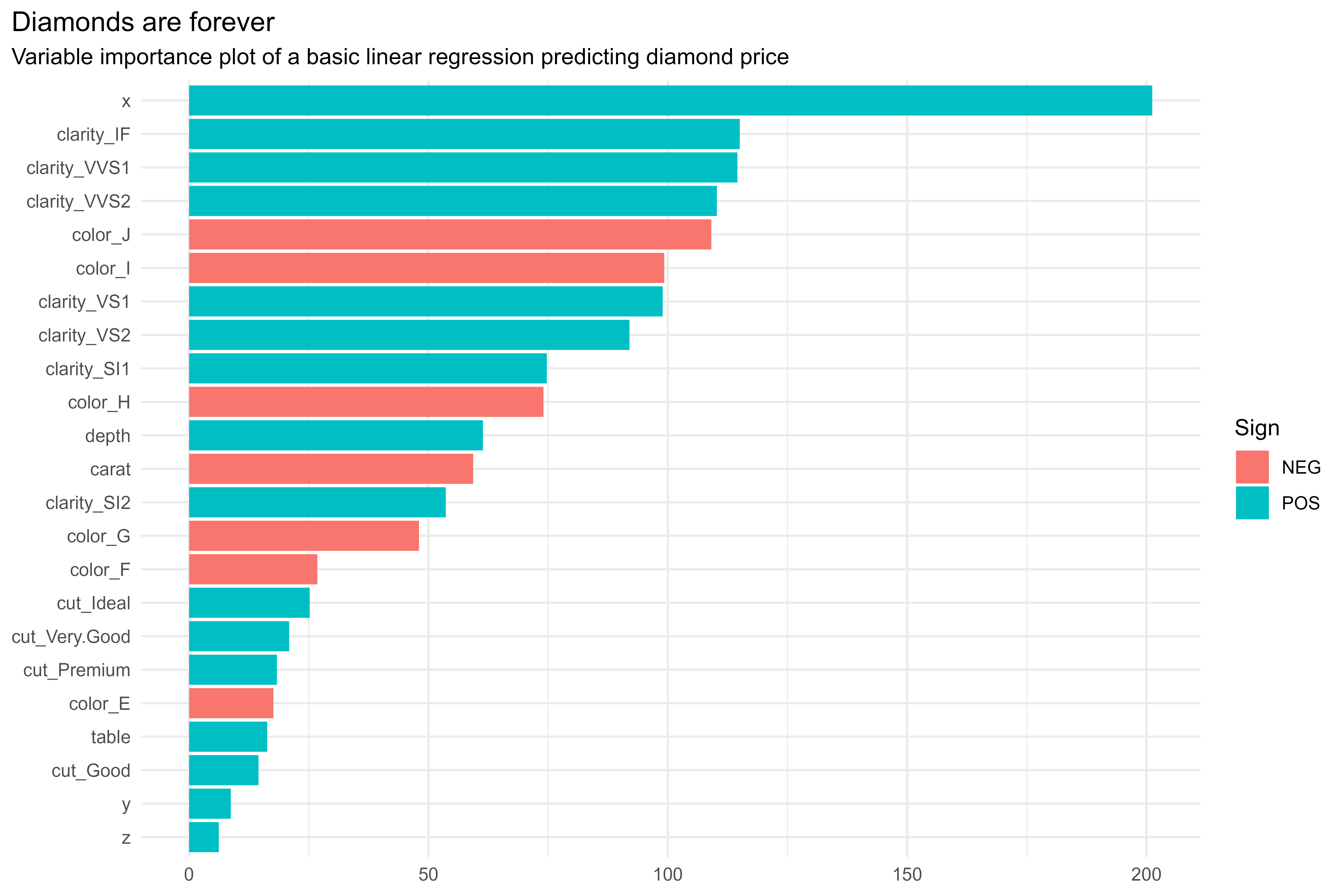Another way of looking at it:

Code
``````set.seed(666)
wf01 %>%
fit(diamonds_train) %>%
pull_workflow_fit() %>%
vip::vi() %>%
mutate(Importance = if_else(Sign == "NEG", Importance * -1, Importance),
Variable = fct_reorder(Variable, Importance)) %>%
ggplot(aes(x = Variable,
y = Importance,
fill = Sign)) +
geom_col() +
coord_flip() +
labs(title = "Diamonds are forever",
subtitle = "Variable importance plot of a basic linear regression predicting diamond price",
x = NULL,
y = NULL) +
theme(plot.title.position = "plot")``````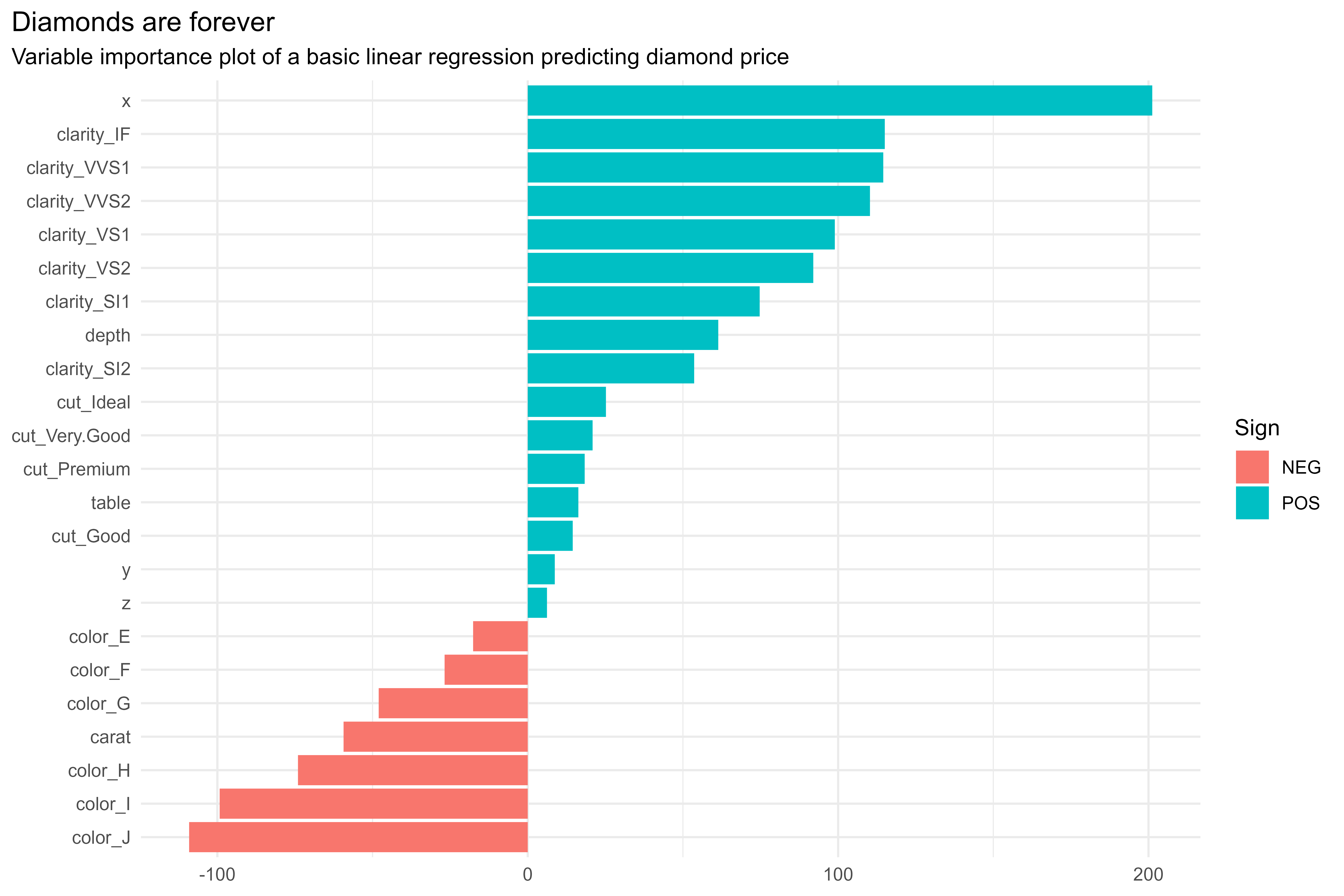This is a good, but definitely improvable, starting point. We can likely decrease our overall error with a bit of feature engineering and drop unimportant features by tuning a regularized model. There are some oddities in this initial model that will need to be improved upon; for one, we can definitively say that the `carat` feature ought to be positively associated with price

Code
``````diamonds_train %>%
ggplot(aes(x = carat,
y = price)) +
geom_point(alpha = 0.01) +
labs(title = "A clear positive (albeit nonlinear) relationship between `carat` and `price`") +
theme(plot.title.position = "plot")``````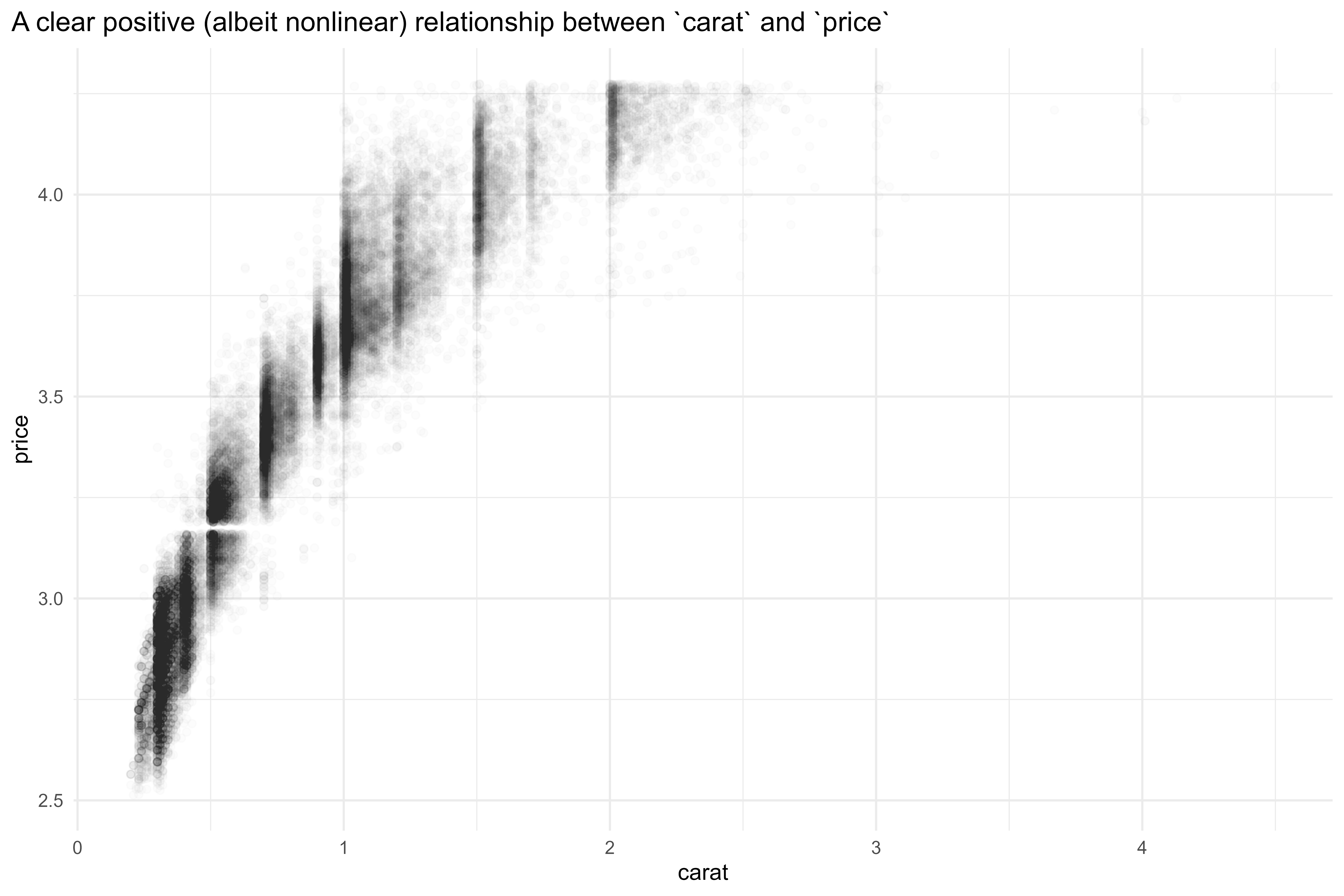Another few things that are interesting to note in this plot! It looks like there are clusterings of carat ratings around round-ish numbers. My hypothesis here is that carat ratings tend to get rounded up to the next size. There’s also a clear abscence of diamonds priced at \$1,500 (~3.17 on the log10 scale). I suppose there is some industry-specific reason to avoid a diamond price of \$,1500?

Code
``````diamonds_train %>%
ggplot(aes(x = carat,
y = price)) +
geom_point(alpha = 0.01) +
labs(title = "A clear positive (albeit nonlinear) relationship between `carat` and `price`") +
theme(plot.title.position = "plot") +
geom_hline(yintercept = log10(1500),
linetype = "dashed",
size = 0.9,
alpha = 0.5)``````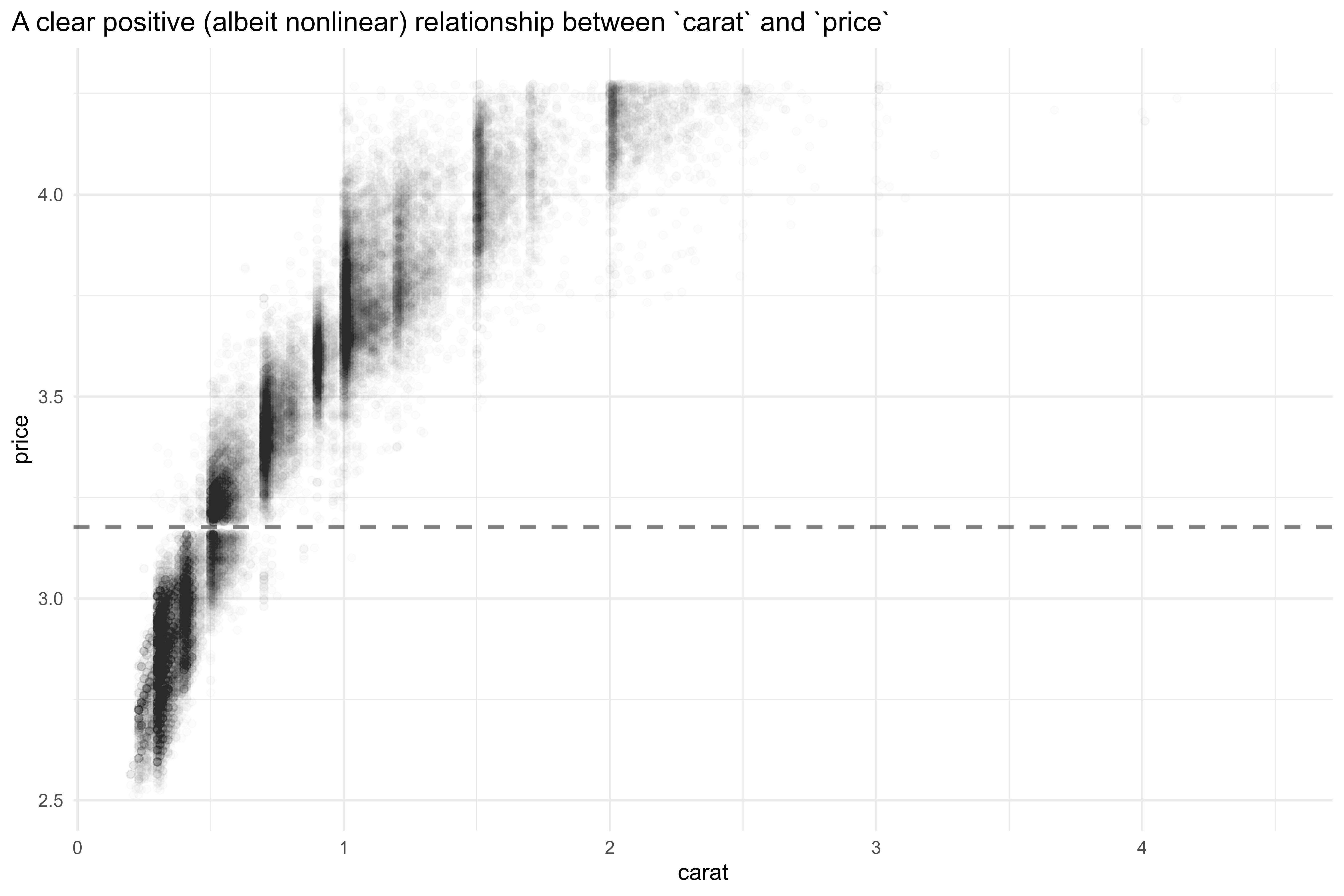How to address all these things? With some feature engineering! Firstly, let’s add some recipe steps to balance classes & normalize continuous variables.

But before I get into that, I’ll save the resample metrics so that we can compare models!

Code
``````metrics <- collect_metrics(rs01) %>% mutate(model = "model01")

metrics``````
``````#> # A tibble: 2 × 7
#>   .metric .estimator   mean     n std_err .config              model
#>   <chr>   <chr>       <dbl> <int>   <dbl> <chr>                <chr>
#> 1 rmse    standard   0.0793    10 0.00557 Preprocessor1_Model1 model01
#> 2 rsq     standard   0.966     10 0.00494 Preprocessor1_Model1 model01``````
Code
``````# spec will be the same as model01
mod02 <- mod01

# recipe!
rec02 <-
recipe(price ~ ., data = diamonds_train) %>%
step_other(cut, color, clarity) %>%
step_dummy(all_nominal_predictors(), -cut) %>%

# use smote resampling to balance classes
themis::step_smote(cut) %>%

# normalize continuous vars
bestNormalize::step_best_normalize(carat, depth, table, x, y, z)``````

Let’s bake our recipe to verify that everything looks up-to-snuff in the preprocessed dataset.

Code
``````baked_rec02 <-
rec02 %>%
prep() %>%
bake(new_data = NULL)

baked_rec02``````
``````#> # A tibble: 80,495 × 20
#>      carat cut        depth  table       x       y      z price color_E color_F
#>      <dbl> <fct>      <dbl>  <dbl>   <dbl>   <dbl>  <dbl> <dbl>   <dbl>   <dbl>
#>  1 -0.706  Premium    0.138 -0.760 -0.709  -0.738  -0.695  3.01       0       1
#>  2  0.356  Very Good  0.570  0.835  0.342   0.251   0.344  3.63       0       0
#>  3  0.214  Premium   -0.308  0.835  0.293   0.263   0.166  3.58       0       0
#>  4 -1.08   other      1.04  -0.310 -1.30   -1.40   -0.995  2.70       0       0
#>  5 -0.641  Ideal     -0.602 -0.760 -0.595  -0.560  -0.622  2.97       0       0
#>  6 -0.0759 Premium   -0.602  0.494 -0.0349 -0.0460 -0.114  3.38       0       0
#>  7 -0.149  Premium   -1.16   0.103 -0.0565 -0.0842 -0.246  3.44       1       0
#>  8  0.170  Very Good -0.371  0.494  0.178   0.313   0.130  3.56       0       1
#>  9 -0.736  Ideal     -0.110 -0.760 -0.709  -0.738  -0.723  3.09       0       0
#> 10  0.782  Ideal     -0.602 -0.310  0.819   0.846   0.732  4.02       0       0
#> # … with 80,485 more rows, and 10 more variables: color_G <dbl>, color_H <dbl>,
#> #   color_I <dbl>, color_J <dbl>, clarity_SI2 <dbl>, clarity_VS1 <dbl>,
#> #   clarity_VS2 <dbl>, clarity_VVS1 <dbl>, clarity_VVS2 <dbl>,
#> #   clarity_other <dbl>``````
Code
``````baked_rec02 %>%
count(cut) %>%
ggplot(aes(x = cut,
y = n)) +
geom_col()``````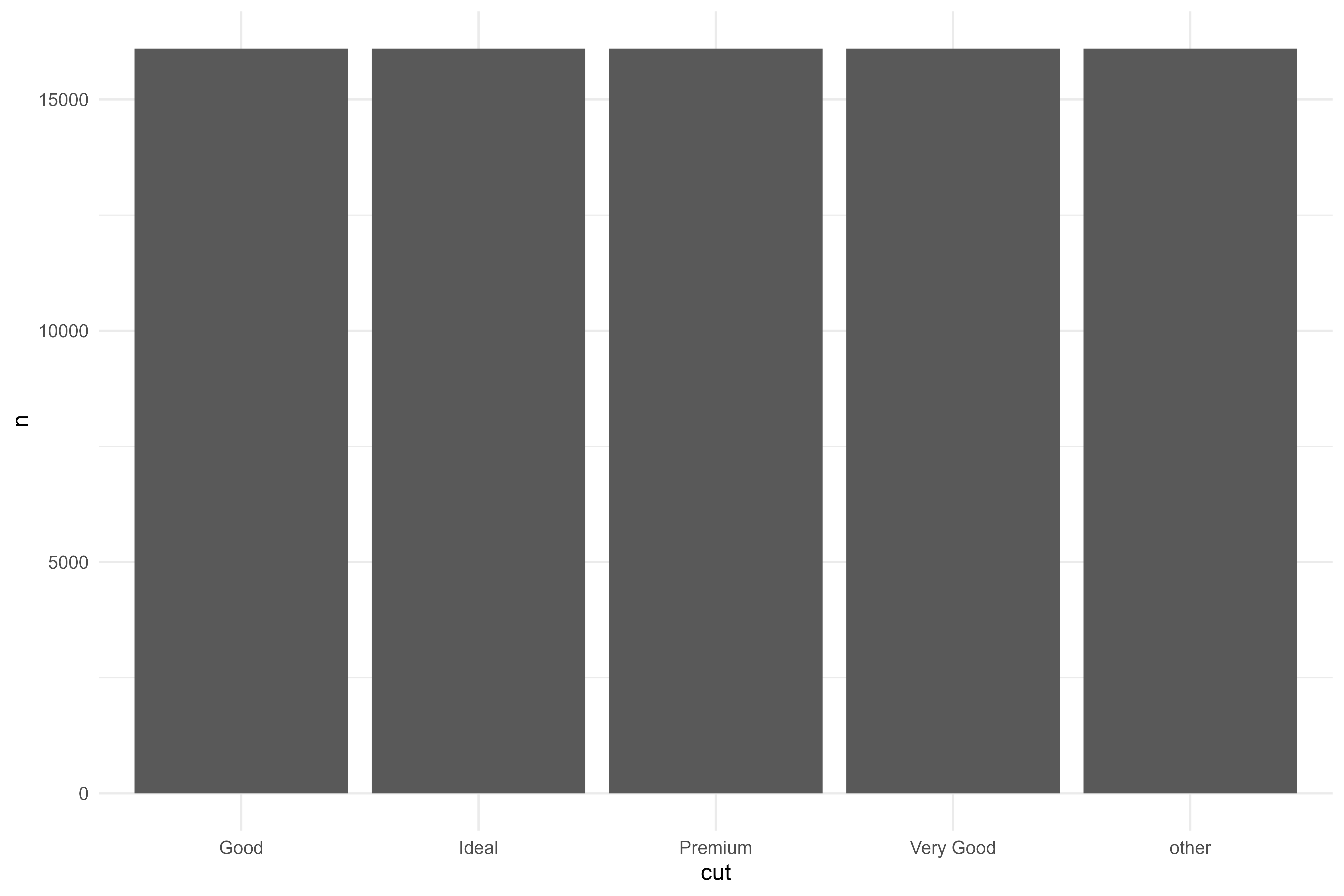Code
``````baked_rec02 %>%
ggplot(aes(x = carat)) +
geom_histogram()``````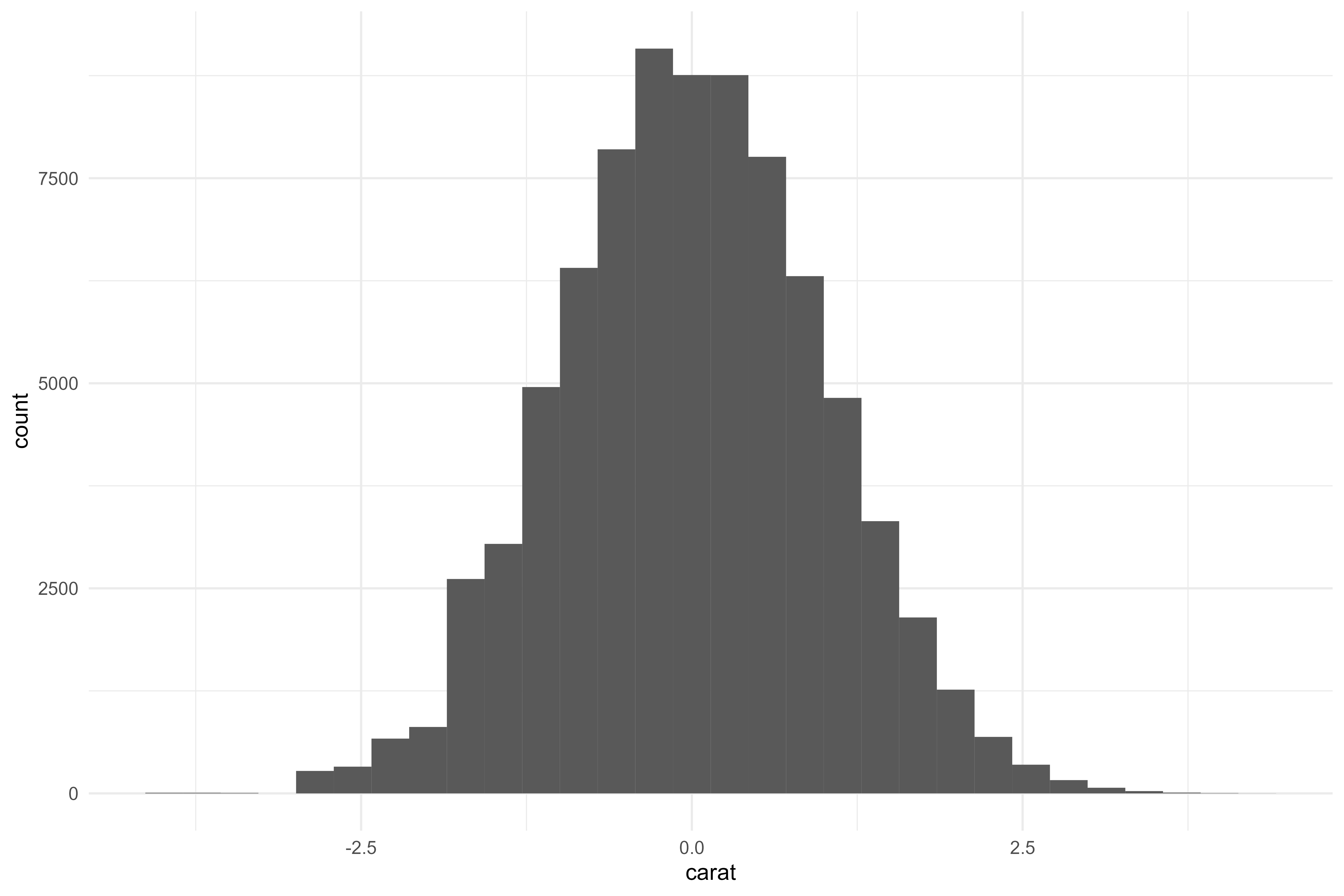Code
``````baked_rec02 %>%
ggplot(aes(x = depth)) +
geom_histogram()``````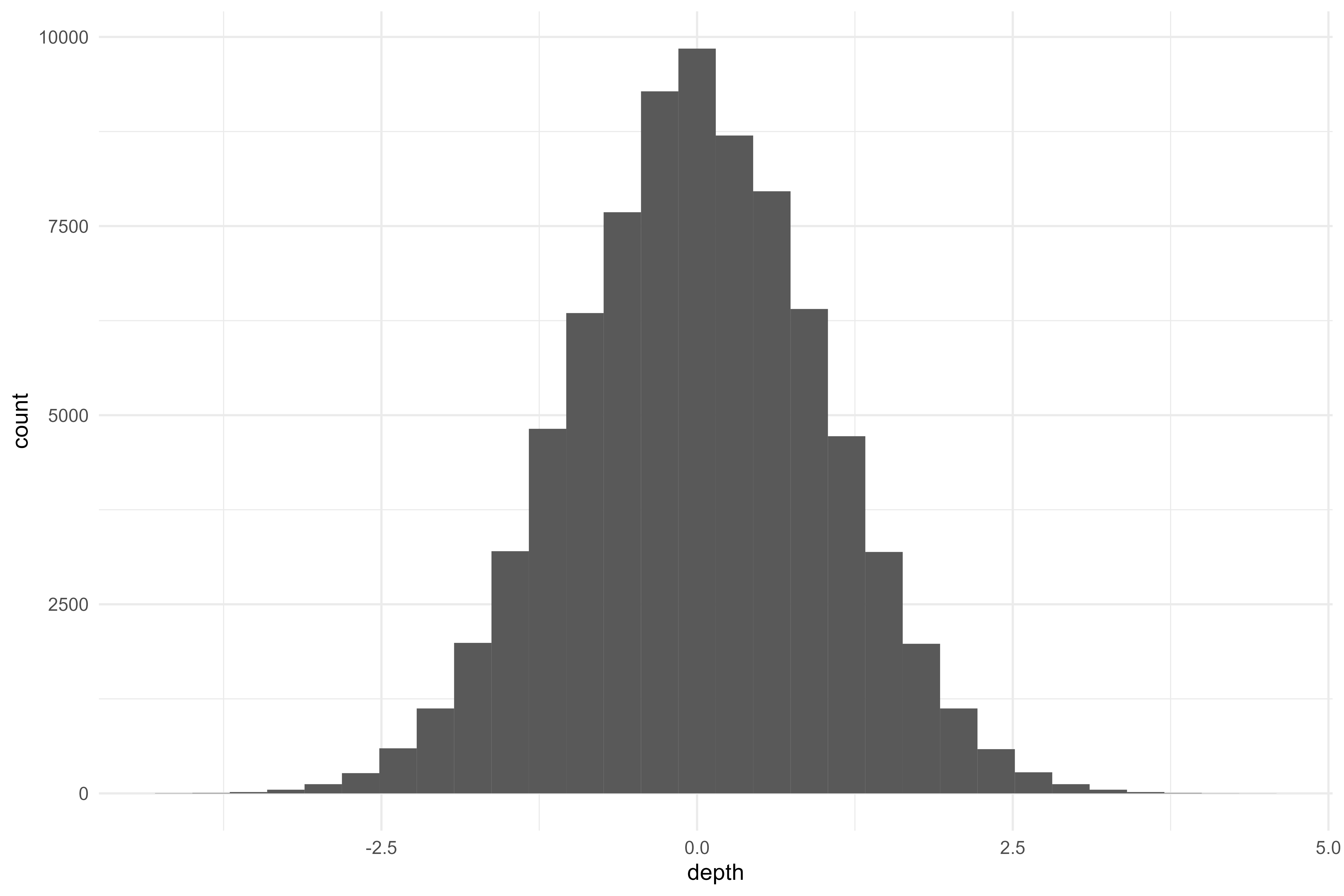Code
``````baked_rec02 %>%
ggplot(aes(x = table)) +
geom_histogram()``````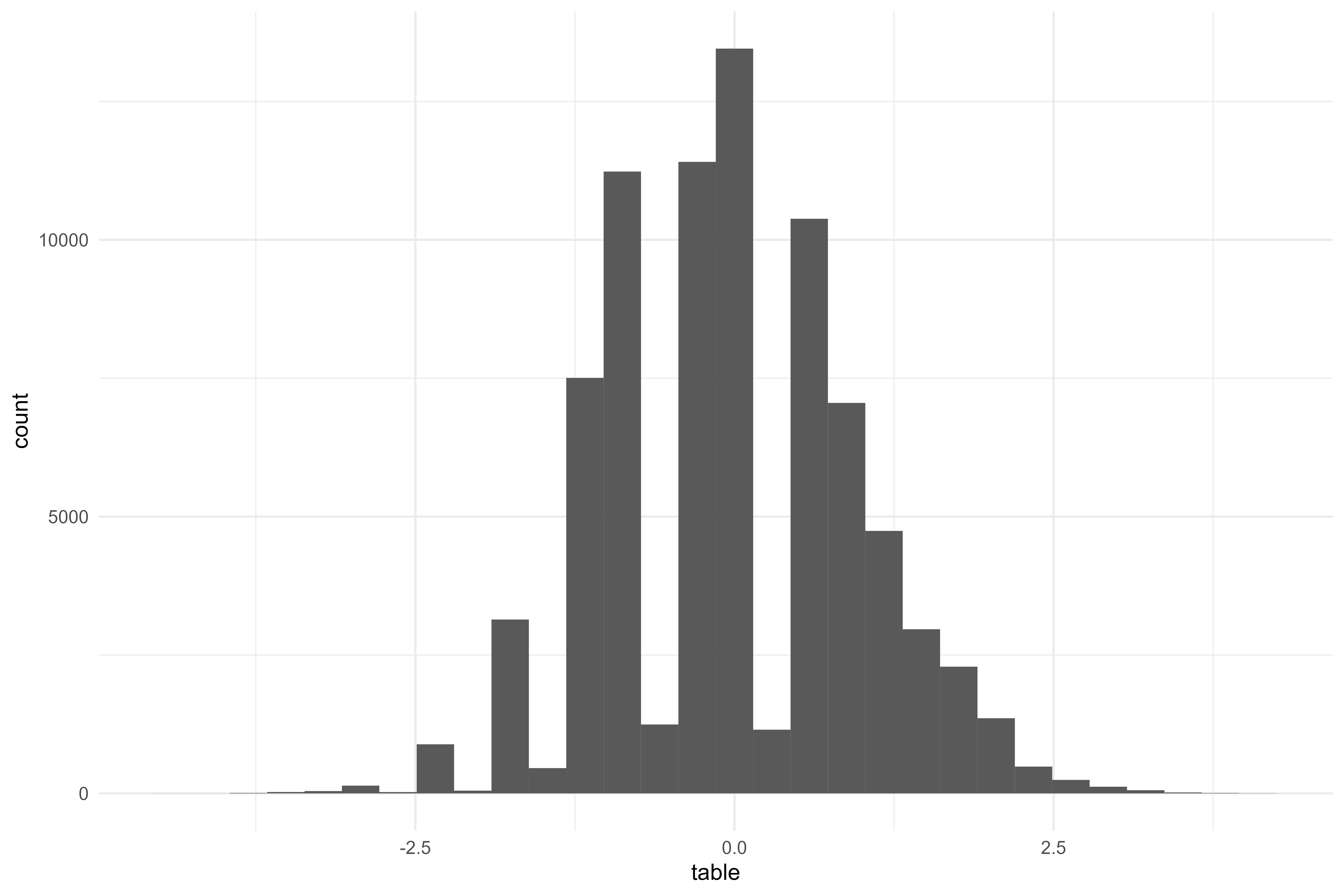Code
``````baked_rec02 %>%
ggplot(aes(x = x)) +
geom_histogram()``````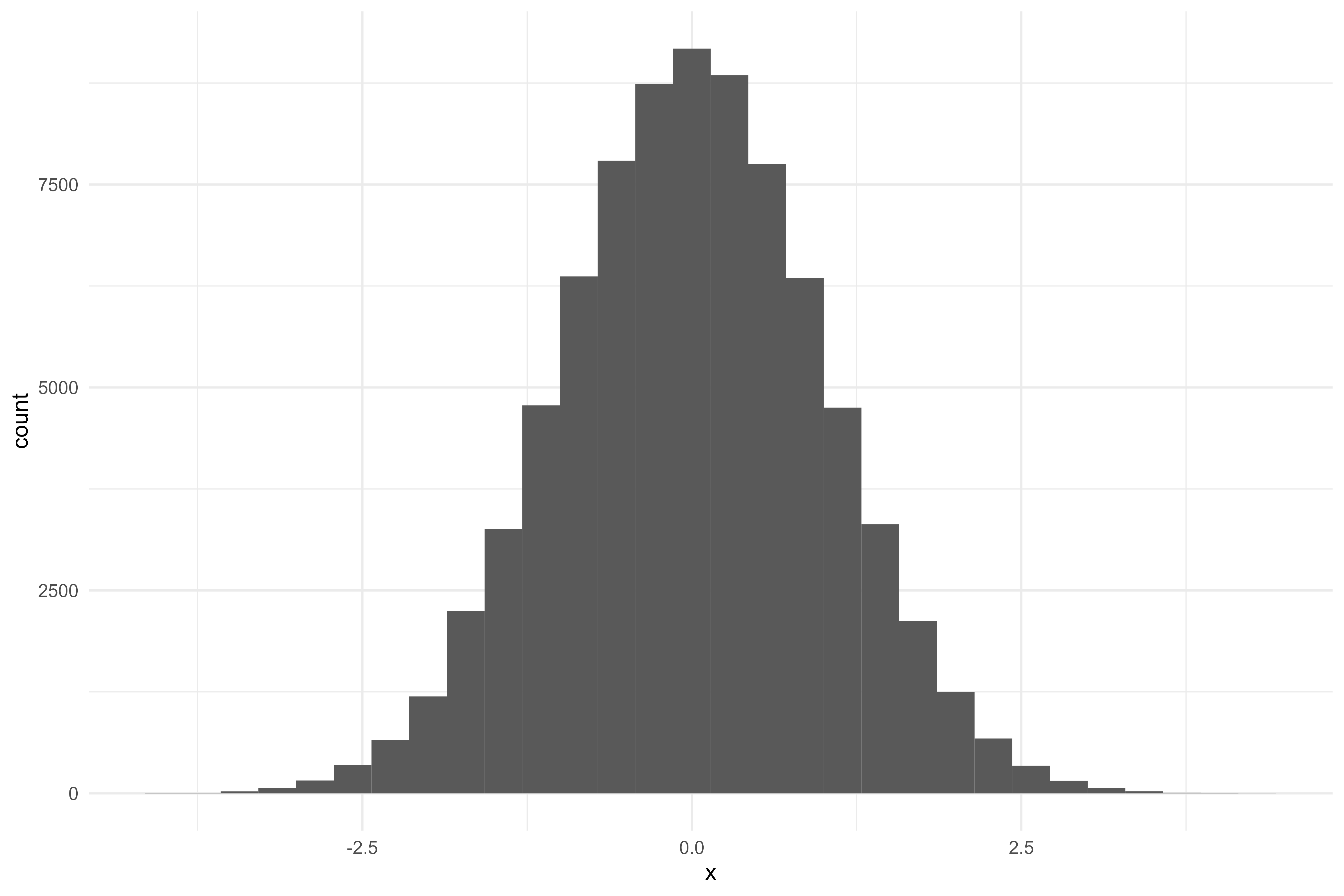Code
``````baked_rec02 %>%
ggplot(aes(x = y)) +
geom_histogram() ``````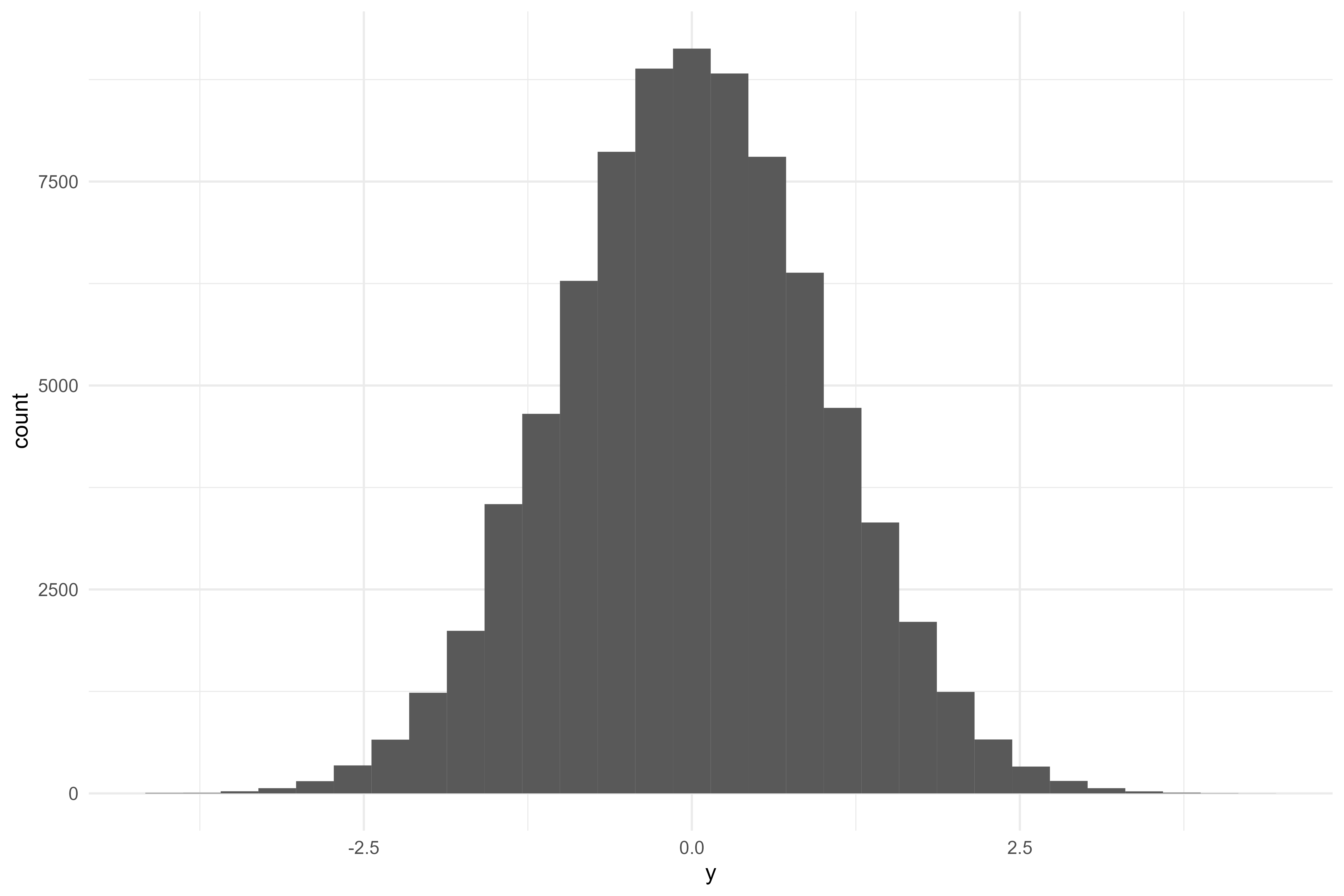Code
``````baked_rec02 %>%
ggplot(aes(x = z)) +
geom_histogram()``````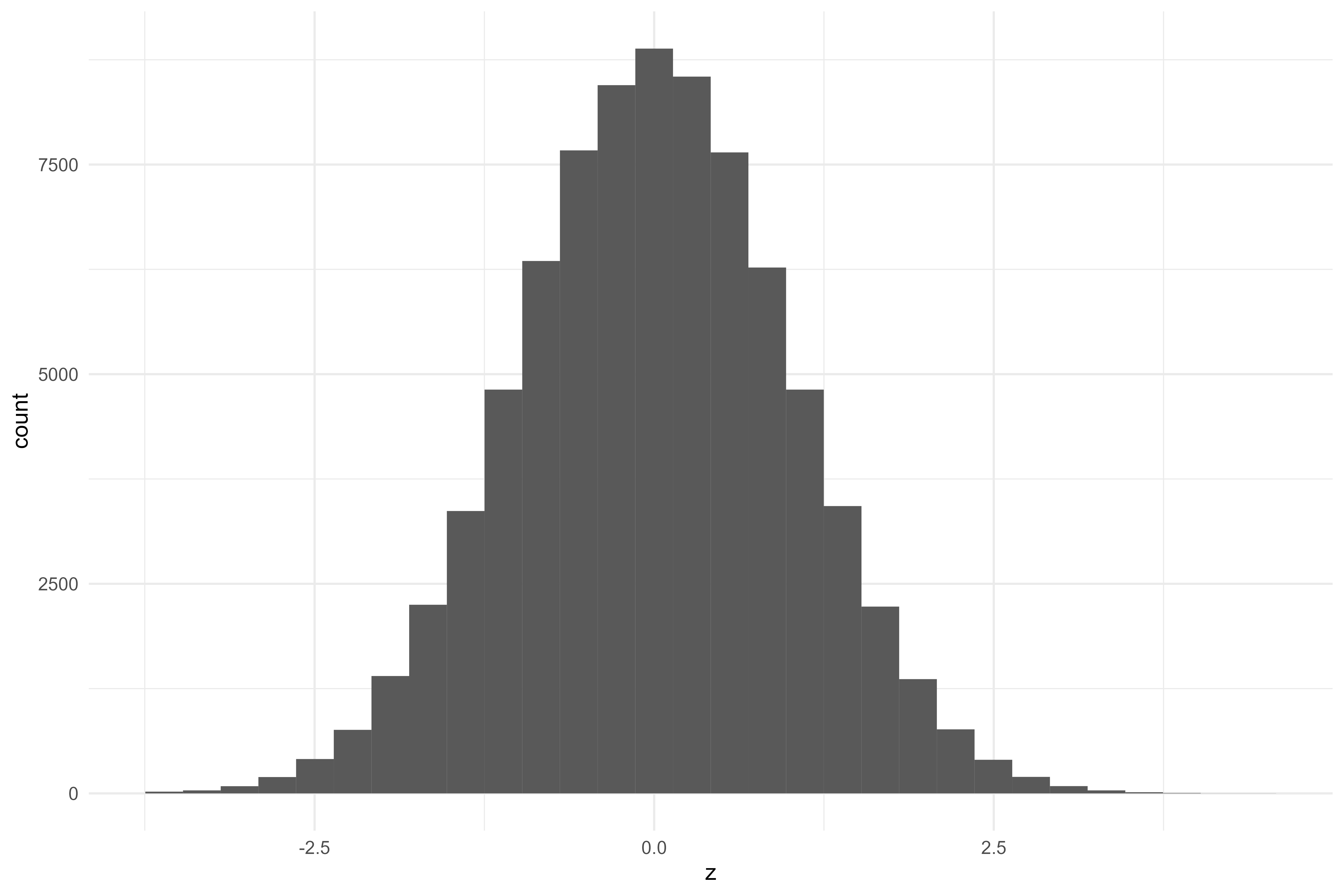Everything looks alright with the exception of the `table` predictor. I wonder if there are a lot of repeated values in the `table` variable - that may be why we’re seeing a “chunky” histogram. Let’s check

Code
``````baked_rec02 %>%
count(table) %>%
arrange(desc(n))``````
``````#> # A tibble: 10,406 × 2
#>     table     n
#>     <dbl> <int>
#>  1  0.103 12167
#>  2 -0.310 11408
#>  3 -0.760 11031
#>  4  0.494  9406
#>  5 -1.28   6726
#>  6  0.835  6165
#>  7  1.15   3810
#>  8 -1.85   2789
#>  9  1.42   2182
#> 10  1.64    972
#> # … with 10,396 more rows``````

Ooh - okay yeah that’s definitely the issue! I’m not quite sure how to deal with it, so we’re just going to ignore for now! Let’s add a new model & see how it compares against the baseline transformed model.

Code
``````wf02 <-
workflow() %>%

# stop parallel to avoid error!
# need to replace with PSOCK clusters
# see github issue here: https://github.com/tidymodels/recipes/issues/847
foreach::registerDoSEQ()

set.seed(666) # spoopy
rs02 <-
fit_resamples(
wf02,
diamonds_folds,
control = ctrl_preds
)

collect_metrics(rs02)``````
``````#> # A tibble: 2 × 6
#>   .metric .estimator  mean     n std_err .config
#>   <chr>   <chr>      <dbl> <int>   <dbl> <chr>
#> 1 rmse    standard   0.115    10 0.00143 Preprocessor1_Model1
#> 2 rsq     standard   0.932    10 0.00161 Preprocessor1_Model1``````

Oof - that’s actually slightly worse than our baseline model!

Code
``````rs02 %>%
augment() %>%
ggplot(aes(x = price,
y = .pred)) +
geom_point(alpha = 0.01) +
geom_abline(linetype = "dashed",
size = 0.1,
alpha = 0.5) ``````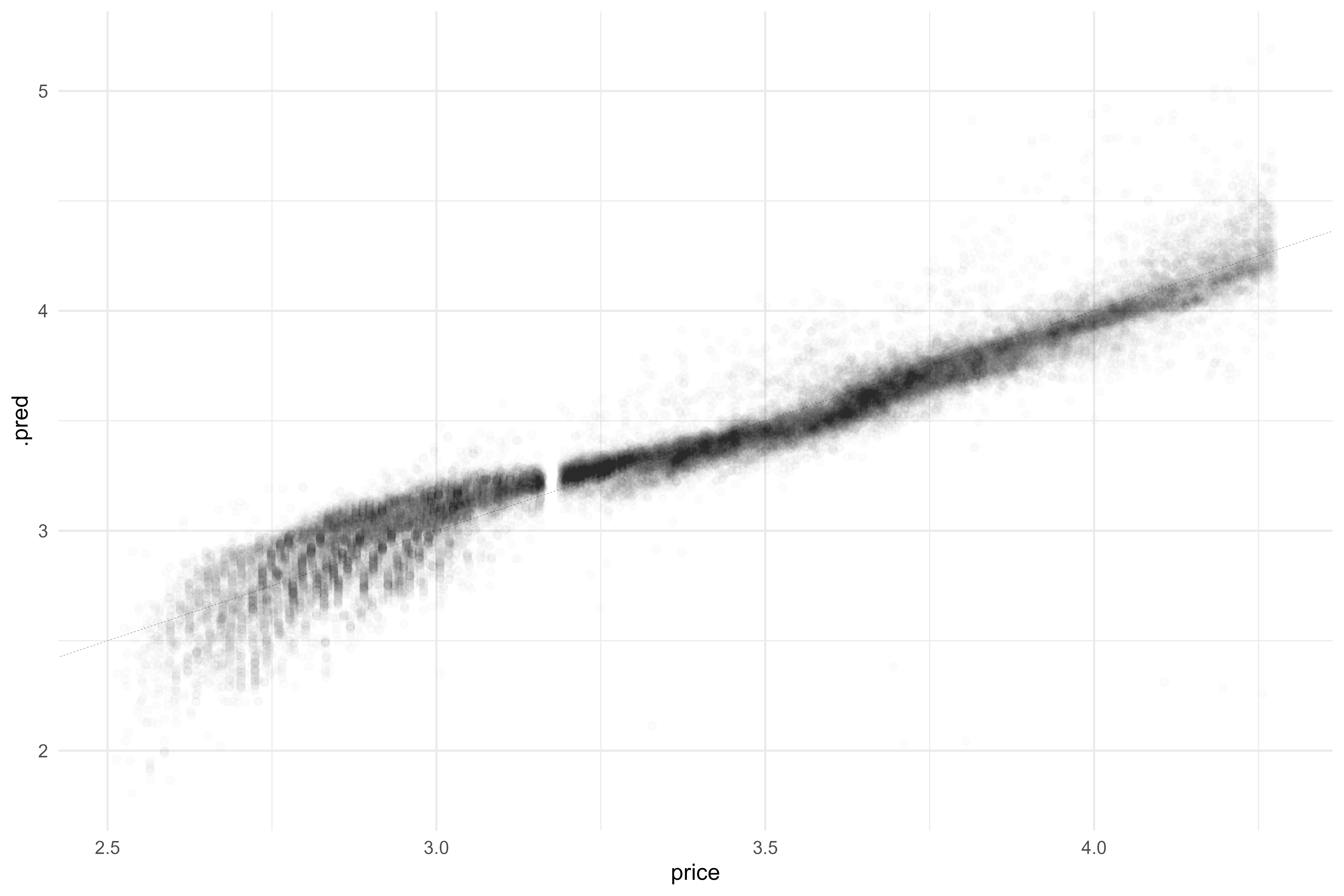It looks like we’ve introduced structure into the residual plot!

Code
``````rs02 %>%
augment() %>%
ggplot(aes(x = price,
y = .resid)) +
geom_point(alpha = 0.01) +
geom_hline(yintercept = 0,
linetype = "dashed",
size = 0.1,
alpha = 0.5)``````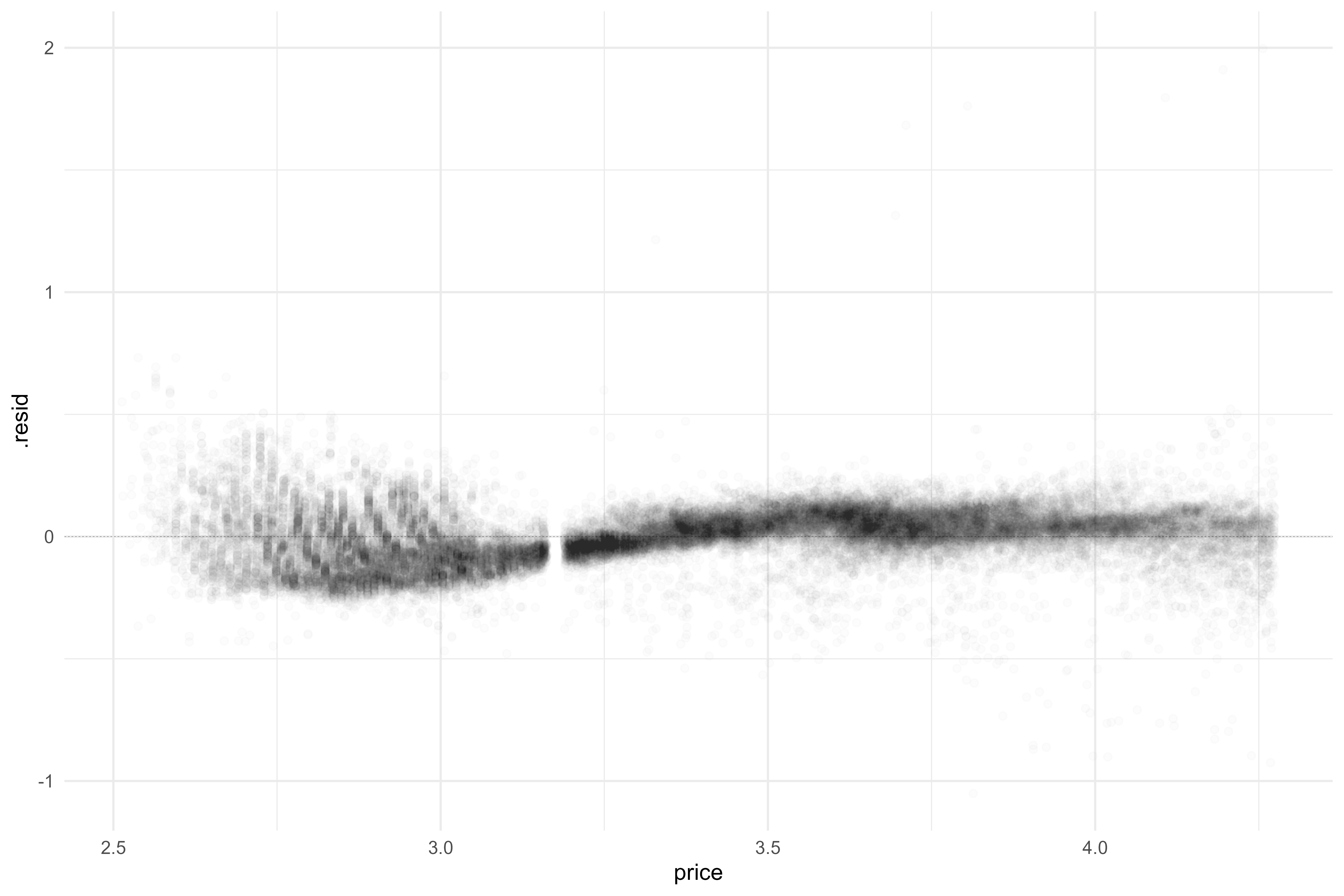Yeah that’s fairly wonky! I’m wondering if it’s due to the SMOTE upsampling method we introduced? To counteract, I’ll build & train new models after each set of recipe steps (e.g., resampling, normalizing, interactions) to buil up a better performing model one step at a time.

Code
``````metrics <-
metrics %>%
bind_rows(collect_metrics(rs02) %>% mutate(model = "model02"))``````
Code
``````# same model spec
mod03 <- mod02

# rebuild rec+wf & retrain
rec03 <-
recipe(price ~ ., data = diamonds_train) %>%
step_other(cut, color, clarity) %>%
step_dummy(all_nominal_predictors(), -cut) %>%
themis::step_smote(cut)

wf03 <-
workflow() %>%

# do paralllel
doParallel::registerDoParallel()

# refit!
set.seed(123)
rs03 <-
fit_resamples(
wf03,
diamonds_folds,
control = ctrl_preds
)

collect_metrics(rs03)``````
``````#> # A tibble: 2 × 6
#>   .metric .estimator   mean     n std_err .config
#>   <chr>   <chr>       <dbl> <int>   <dbl> <chr>
#> 1 rmse    standard   0.0918    10 0.00502 Preprocessor1_Model1
#> 2 rsq     standard   0.956     10 0.00502 Preprocessor1_Model1``````

Interesting! Improved relative to `rs02`, but still not as good as our first model! Let’s try using `step_downsample()` to balance classes & see how we fare.

Code
``````# cleanup some large-ish items eating up memory
rm(mod01, mod02, rec01, rec02, wf01, wf02, rs01, rs02)

# save metrics
metrics <-
metrics %>%
bind_rows(collect_metrics(rs03) %>% mutate(model = "model03"))

# new mod
mod04 <- mod03

# new rec
rec04 <-
recipe(price ~ ., data = diamonds_train) %>%
step_other(cut, color, clarity) %>%
step_dummy(all_nominal_predictors(), -cut) %>%
themis::step_downsample(cut)

wf04 <-
workflow() %>%

set.seed(456)
rs04 <-
fit_resamples(
wf04,
diamonds_folds,
control = ctrl_preds
)

collect_metrics(rs04)``````
``````#> # A tibble: 2 × 6
#>   .metric .estimator  mean     n std_err .config
#>   <chr>   <chr>      <dbl> <int>   <dbl> <chr>
#> 1 rmse    standard   0.113    10  0.0120 Preprocessor1_Model1
#> 2 rsq     standard   0.930    10  0.0153 Preprocessor1_Model1``````

Wow - still a bit worse! I’ll try upsampling & if there is no improvement, we’ll move on without resampling!

Code
``````metrics <-
metrics %>%
bind_rows(collect_metrics(rs04) %>% mutate(model = "model04"))

mod05 <- mod04

rec05 <-
recipe(price ~ ., data = diamonds_train) %>%
step_other(cut, color, clarity) %>%
step_dummy(all_nominal_predictors(), -cut) %>%
themis::step_upsample(cut)

wf05 <-
workflow() %>%

set.seed(789)
rs05 <-
fit_resamples(
wf05,
diamonds_folds,
control = ctrl_preds
)

collect_metrics(rs05)``````
``````#> # A tibble: 2 × 6
#>   .metric .estimator  mean     n std_err .config
#>   <chr>   <chr>      <dbl> <int>   <dbl> <chr>
#> 1 rmse    standard   0.101    10 0.00486 Preprocessor1_Model1
#> 2 rsq     standard   0.946    10 0.00527 Preprocessor1_Model1``````

Okay - resampling gets stricken off our list of recipe steps! Let’s look at how the models compare so far

Code
``````metrics <-
metrics %>%
bind_rows(collect_metrics(rs05) %>% mutate(model = "model05"))

metrics %>%
ggplot(aes(x = model)) +
geom_point(aes(y = mean)) +
geom_errorbar(aes(ymin = mean - std_err,
ymax = mean + std_err)) +
facet_wrap(~.metric, scales = "free_y")``````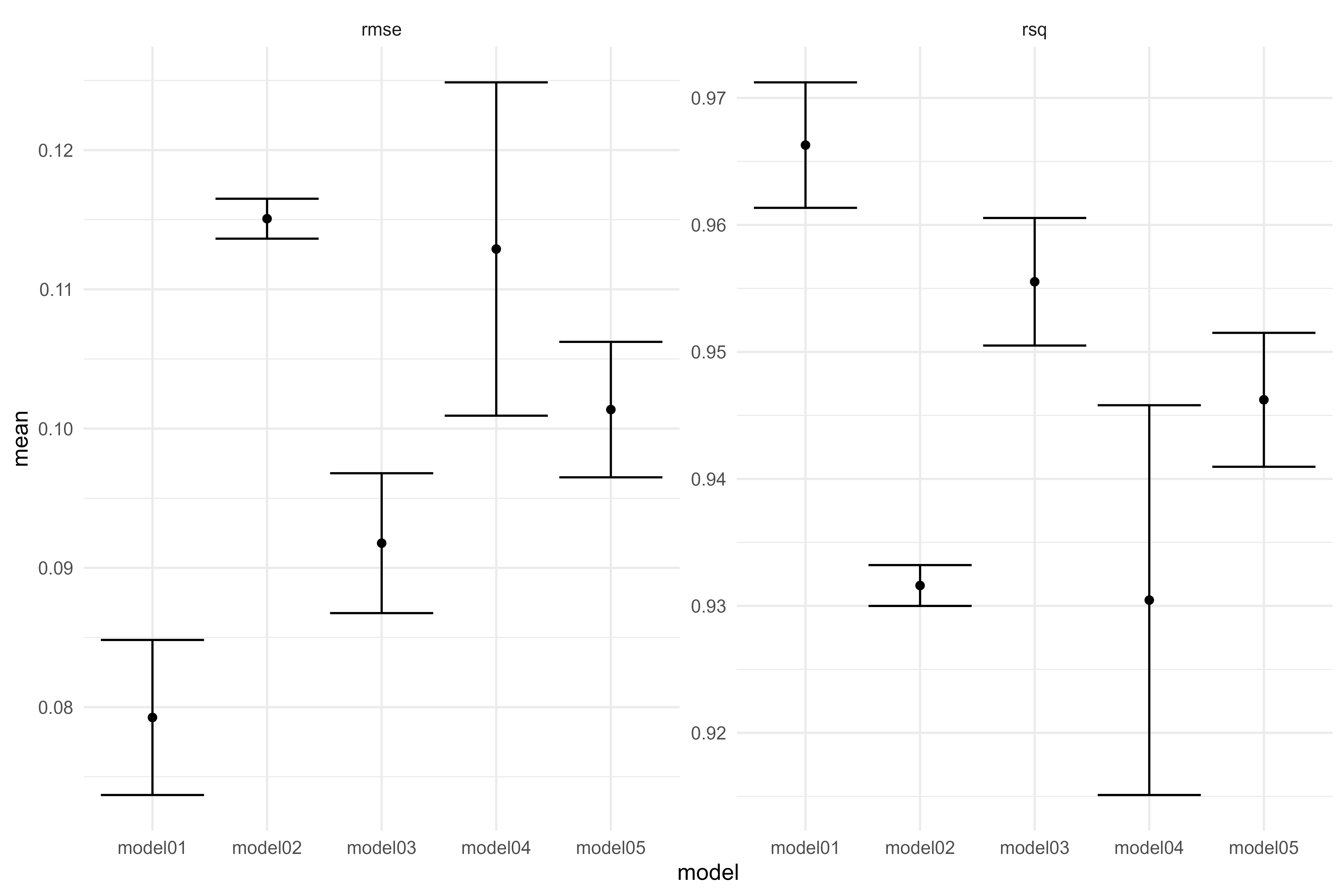The first simple linear model was the best as measured by both metrics! Let’s see if we can improve with some normalization of the continuous vars.

Code
``````rm(mod03, mod04, rec03, rec04, rs03, rs04, wf03, wf04)

mod06 <- mod05

rec06 <-
recipe(price ~ ., data = diamonds_train) %>%
step_other(cut, color, clarity) %>%
bestNormalize::step_best_normalize(all_numeric_predictors()) %>%
step_dummy(all_nominal_predictors())

wf06 <-
workflow() %>%

foreach::registerDoSEQ()
set.seed(101112)
rs06 <-
fit_resamples(
wf06,
diamonds_folds,
control = ctrl_preds
)

collect_metrics(rs06)``````
``````#> # A tibble: 2 × 6
#>   .metric .estimator  mean     n std_err .config
#>   <chr>   <chr>      <dbl> <int>   <dbl> <chr>
#> 1 rmse    standard   0.127    10 0.00115 Preprocessor1_Model1
#> 2 rsq     standard   0.916    10 0.00136 Preprocessor1_Model1``````

Well - that was quite a bit for no improvement! I guess that normalizing the continuous vars in this case isn’t helping. Moving on to adding some interactions - first let’s explore potential interactions a bit.

Code
``````metrics <-
metrics %>%
bind_rows(collect_metrics(rs06) %>% mutate(model = "model06"))

diamonds_train %>%
ggplot(aes(x = carat,
y = price,
color = cut)) +
geom_point(alpha = 0.05) +
geom_smooth(method = "lm", se = FALSE)``````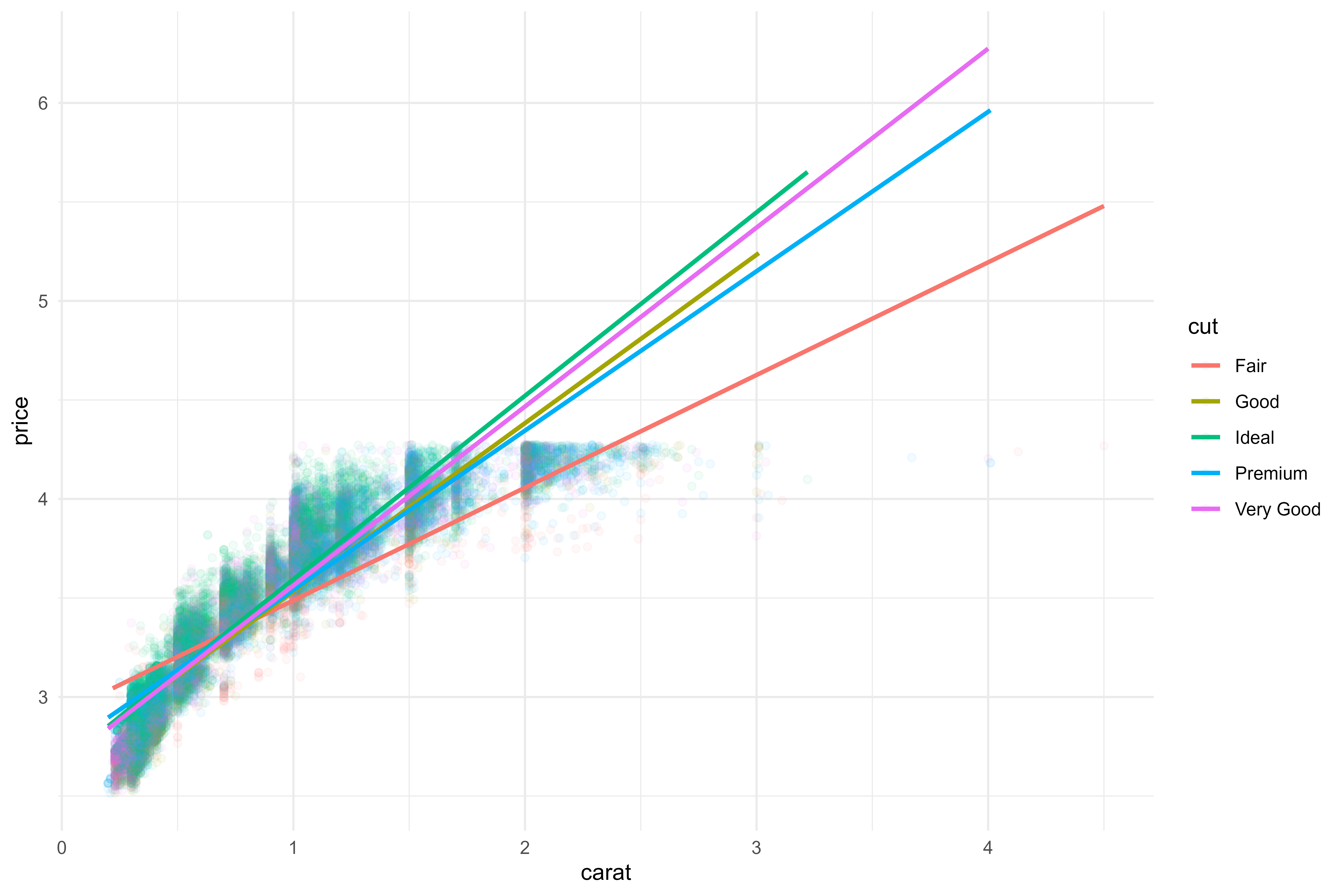Code
``````library(splines)
diamonds_train %>%
ggplot(aes(x = carat,
y = price,
color = cut)) +
geom_point(alpha = 0.05) +
geom_smooth(method = lm,
formula = y ~ ns(x, df = 5),
se = FALSE) +
facet_wrap(~cut, scales = "free")``````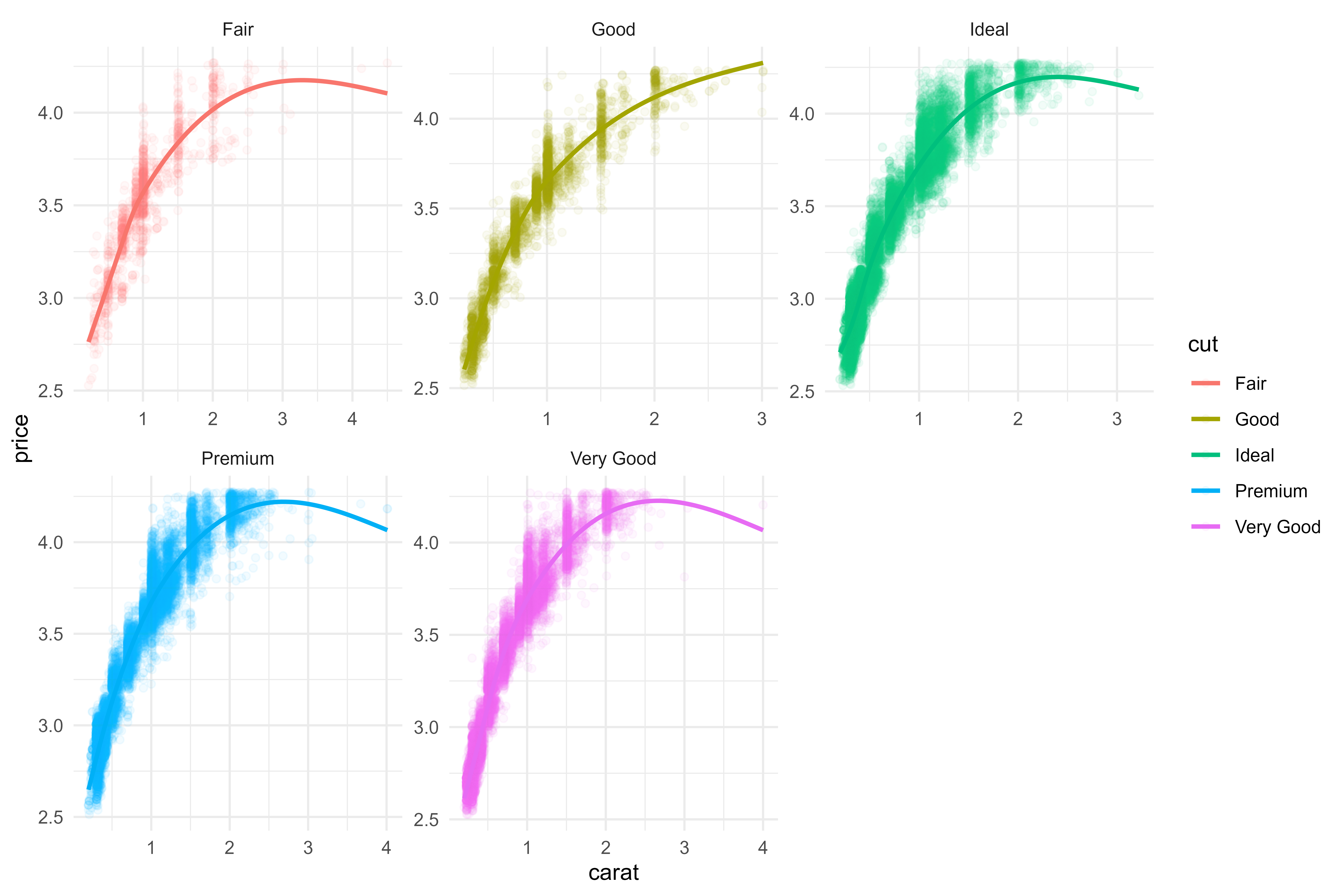5 spline terms might not be sufficient here - capturing the lower bound well but really not doing well with the higher carat diamonds.

Code
``````diamonds_train %>%
ggplot(aes(x = carat,
y = price,
color = cut)) +
geom_point(alpha = 0.05) +
geom_smooth(method = lm,
formula = y ~ ns(x, df = 10),
se = FALSE) +
facet_wrap(~cut, scales = "free")``````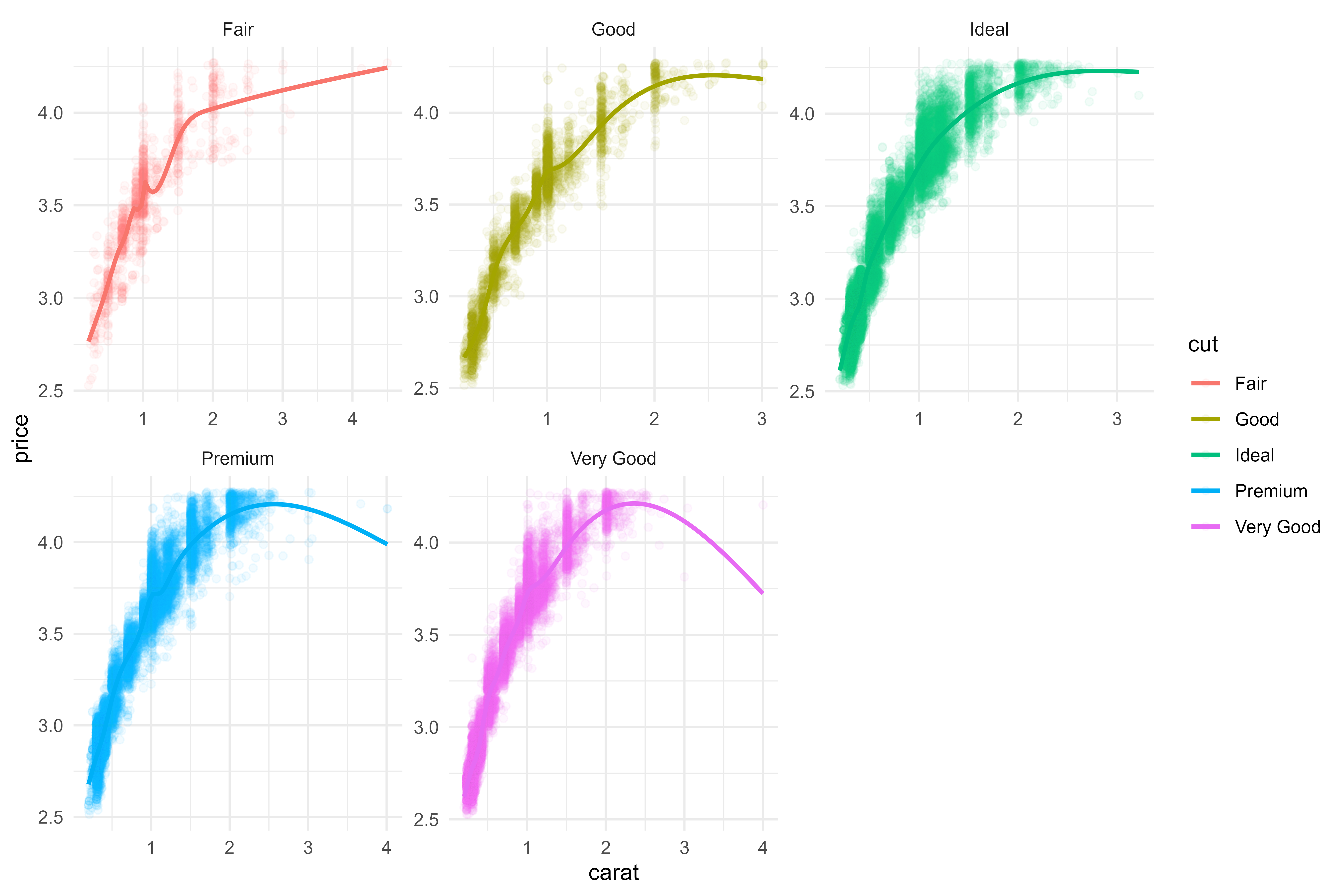Hmmmm, 10 might be too many. It looks lie we’ll just lose a bit of confidence for the Premium & Very Good diamonds at higher carats. Relative to the total number, I’m not too concerned.

Code
``````diamonds_train %>%
ggplot(aes(x = carat,
y = price,
color = cut)) +
geom_point(alpha = 0.05) +
geom_smooth(method = lm,
formula = y ~ ns(x, df = 7),
se = FALSE) +
facet_wrap(~cut, scales = "free")``````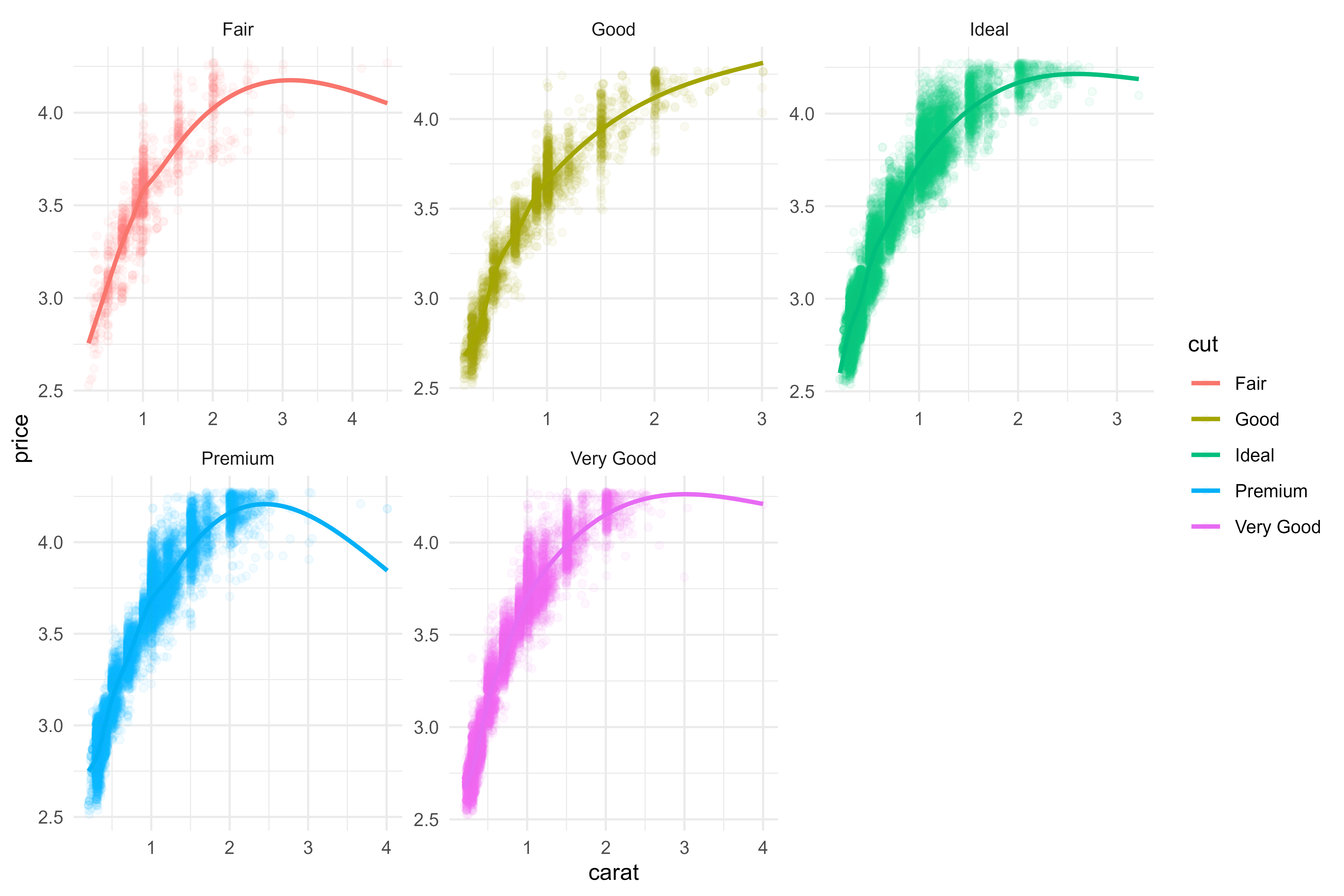7 terms feels like the best we’re going to do here - I think this is tuneable, but we’ll leave as is (now & in the final model).

Next, we’ll look at creating interactions between the `color` and `carat` variables:

Code
``````diamonds_train %>%
ggplot(aes(x = carat,
y = price,
color = color)) +
geom_point(alpha = 0.05) +
geom_smooth(method = lm,
formula = y ~ ns(x, df = 15),
se = FALSE) +
facet_wrap(~color)``````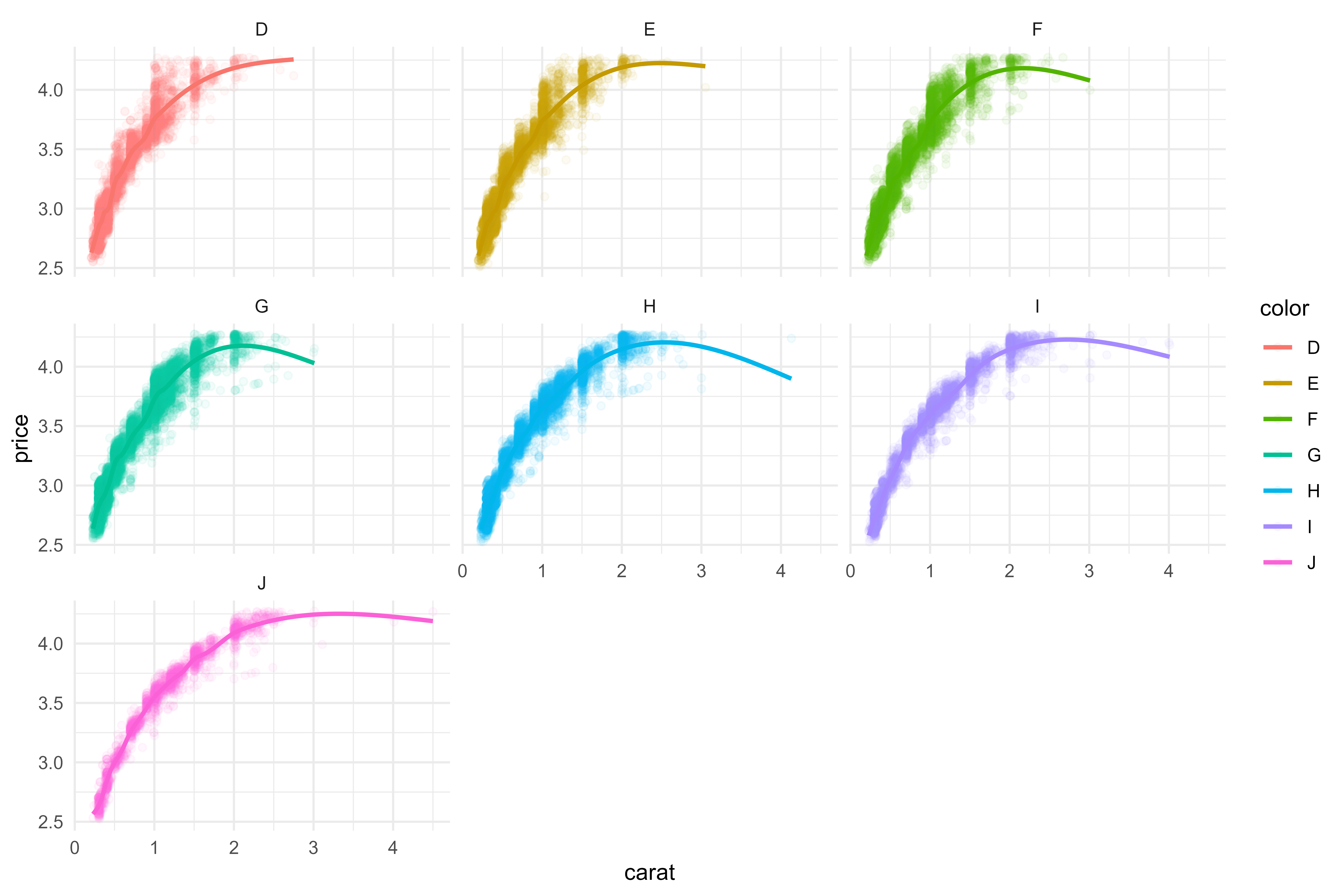Adding interactive spline terms with `df` of 15 seems to add some useful information!

We have three shape parameters, `x`, `y`, and `z` - I wonder if creating a stand-in for volume by multiplying them all together will provide any useful information?

Code
``````diamonds_train %>%
mutate(volume_param = x * y * z) %>%
ggplot(aes(x = volume_param,
y = price)) +
geom_point(alpha = 0.05)``````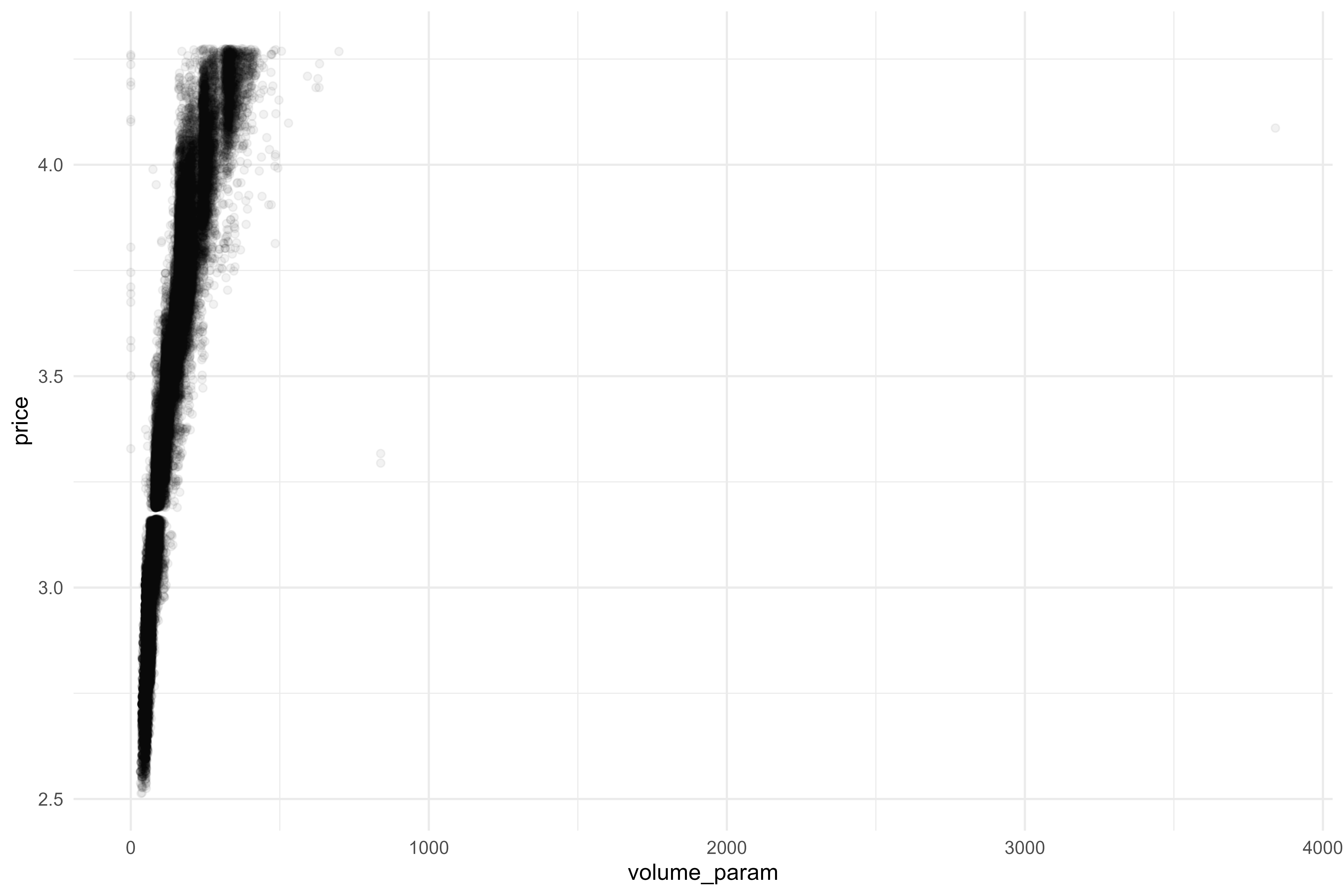Ooh, looks like we’re getting some good info here, but we may want to use `log10` to scale this back.

Code
``````diamonds_train %>%
mutate(volume_param = log10(x * y * z)) %>%
ggplot(aes(x = volume_param,
y = price)) +
geom_point(alpha = 0.05)``````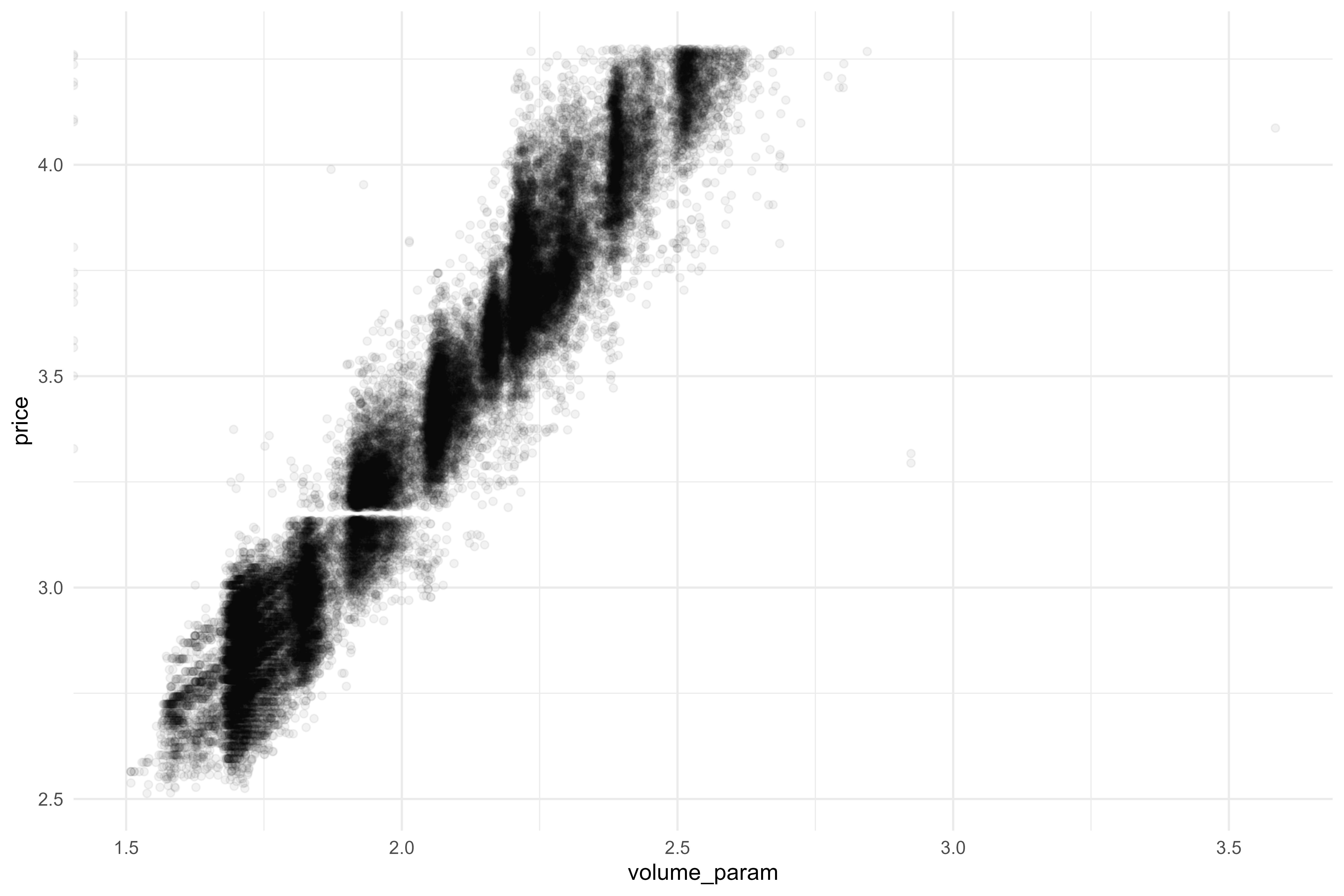Let’s see if this ought to interact with any other paramaters:

Code
``````diamonds_train %>%
mutate(volume_param = log10(x * y * z)) %>%
ggplot(aes(x = volume_param,
y = price,
color = cut)) +
geom_point(alpha = 0.05) +
geom_smooth(method = "lm", se = FALSE)``````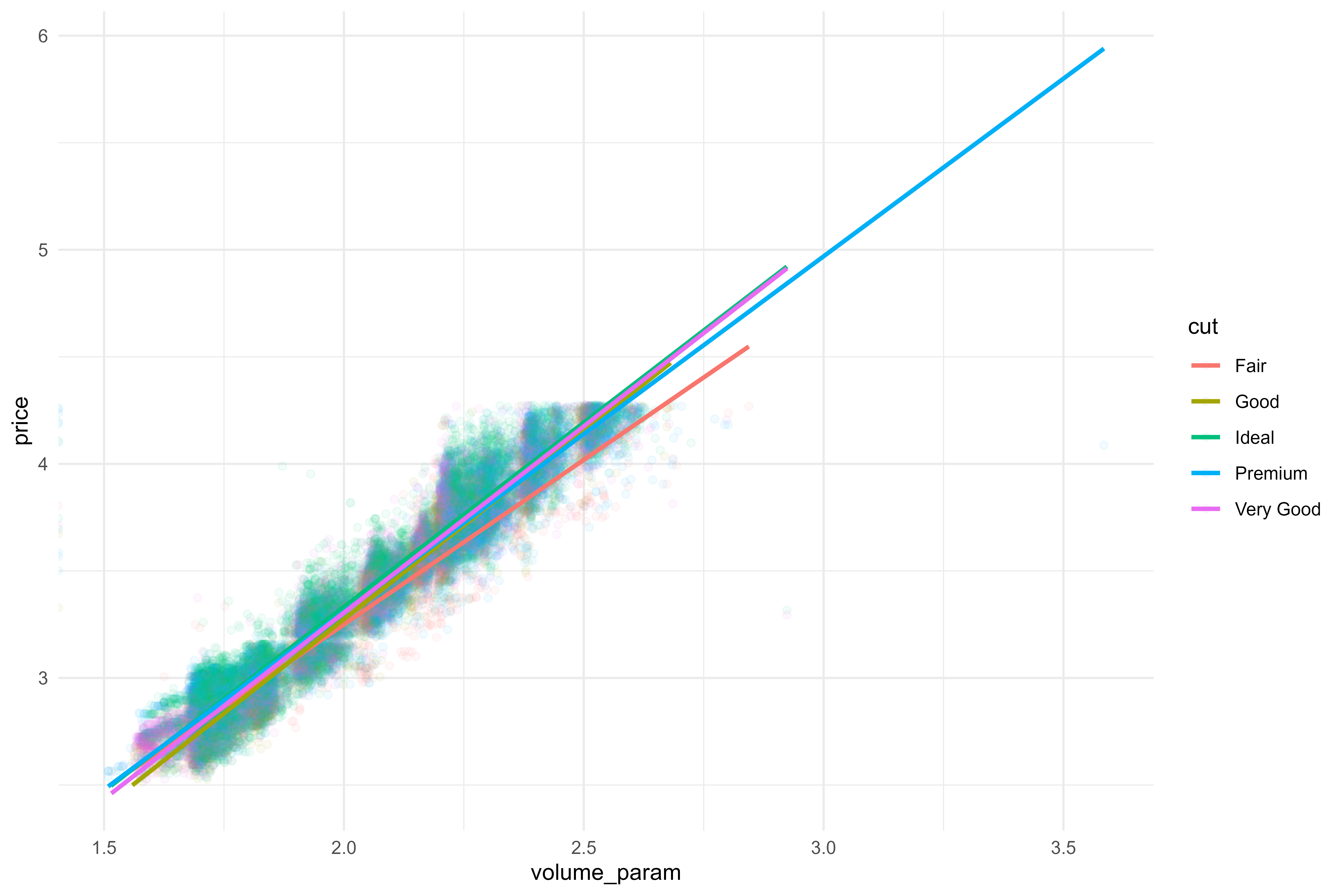Code
``````diamonds_train %>%
mutate(volume_param = log10(x * y * z)) %>%
ggplot(aes(x = volume_param,
y = price,
color = color)) +
geom_point(alpha = 0.05) +
geom_smooth(method = "lm", se = FALSE)``````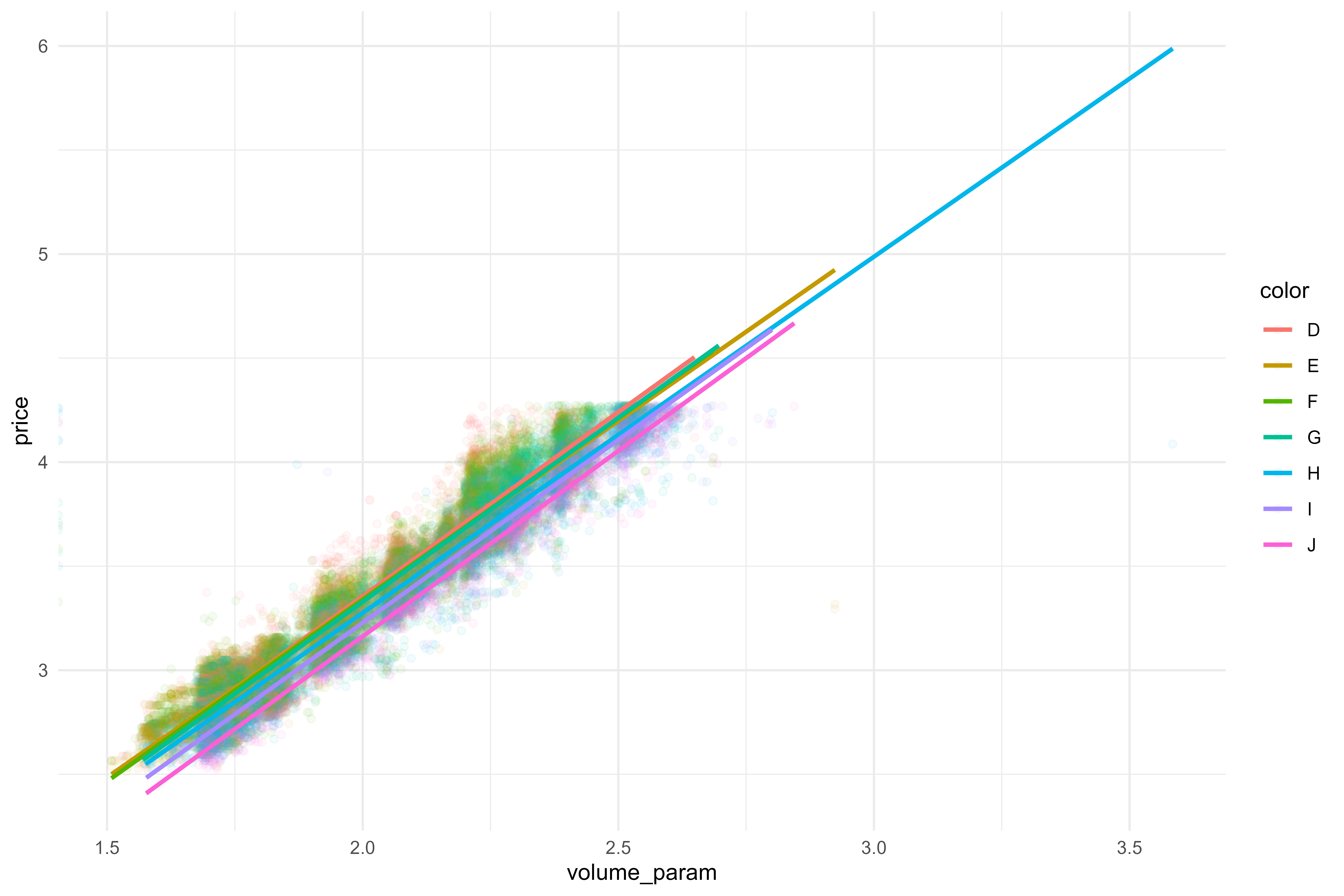Code
``````diamonds_train %>%
mutate(volume_param = log10(x * y * z)) %>%
ggplot(aes(x = volume_param,
y = price,
color = clarity)) +
geom_point(alpha = 0.05) +
geom_smooth(method = "lm", se = FALSE)``````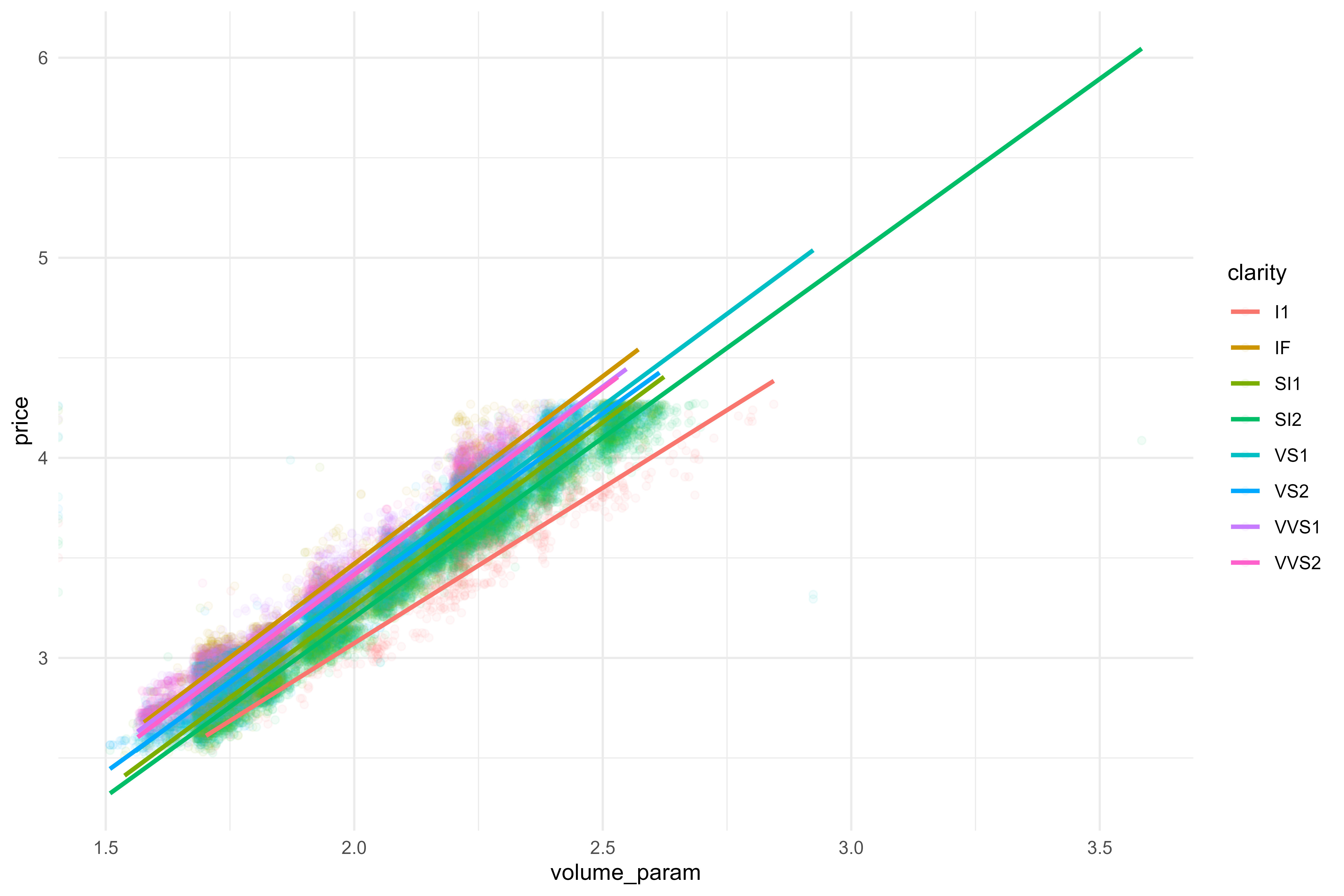Hmm, it doesn’t really look like we’re capturing too great of interactions, so I’ll leave out for now. It looks like the size of the rock is more important than anything else! I could continue to dig further, but I’ll stop there. I’m likely getting diminishing returns, & I’d like to get back into modeling!

Code
``````mod07 <- mod06

rec07 <-
recipe(price ~ ., data = diamonds_train) %>%
step_other(cut, color, clarity) %>%
step_dummy(all_nominal_predictors()) %>%
step_interact(~carat:starts_with("cut_")) %>%
step_interact(~carat:starts_with("color_")) %>%
step_mutate_at(c(x, y, z),
fn = ~if_else(.x == 0, mean(.x), .x)) %>%
step_mutate(volume_param = log10(x * y * z)) %>%
step_ns(starts_with("carat_x_cut"), deg_free = 7) %>%
step_ns(starts_with("carat_x_color"), deg_free = 15)

rec07``````
``````#> Recipe
#>
#> Inputs:
#>
#>       role #variables
#>    outcome          1
#>  predictor          9
#>
#> Operations:
#>
#> Collapsing factor levels for cut, color, clarity
#> Dummy variables from all_nominal_predictors()
#> Interactions with carat:starts_with("cut_")
#> Interactions with carat:starts_with("color_")
#> Variable mutation for c(x, y, z)
#> Variable mutation for log10(x * y * z)
#> Natural splines on starts_with("carat_x_cut")
#> Natural splines on starts_with("carat_x_color")``````
Code
``````wf07 <-
workflow() %>%

doParallel::registerDoParallel()
set.seed(9876)
rs07 <-
fit_resamples(
wf07,
diamonds_folds,
control = ctrl_preds
)``````

This is definitely going to way overfit our data:

Code
``````rs07 %>%
collect_metrics()``````
``````#> # A tibble: 2 × 6
#>   .metric .estimator   mean     n std_err .config
#>   <chr>   <chr>       <dbl> <int>   <dbl> <chr>
#> 1 rmse    standard   0.0750    10 0.00377 Preprocessor1_Model1
#> 2 rsq     standard   0.970     10 0.00342 Preprocessor1_Model1``````

Well we (finally) made a modes improvement! Let’s see how the predictions/residuals plot:

Code
``````rs07 %>%
augment() %>%
ggplot(aes(x = price,
y = .pred)) +
geom_point(alpha = 0.05) +
geom_abline(linetype = "dashed",
alpha = 0.5,
size = 0.5)``````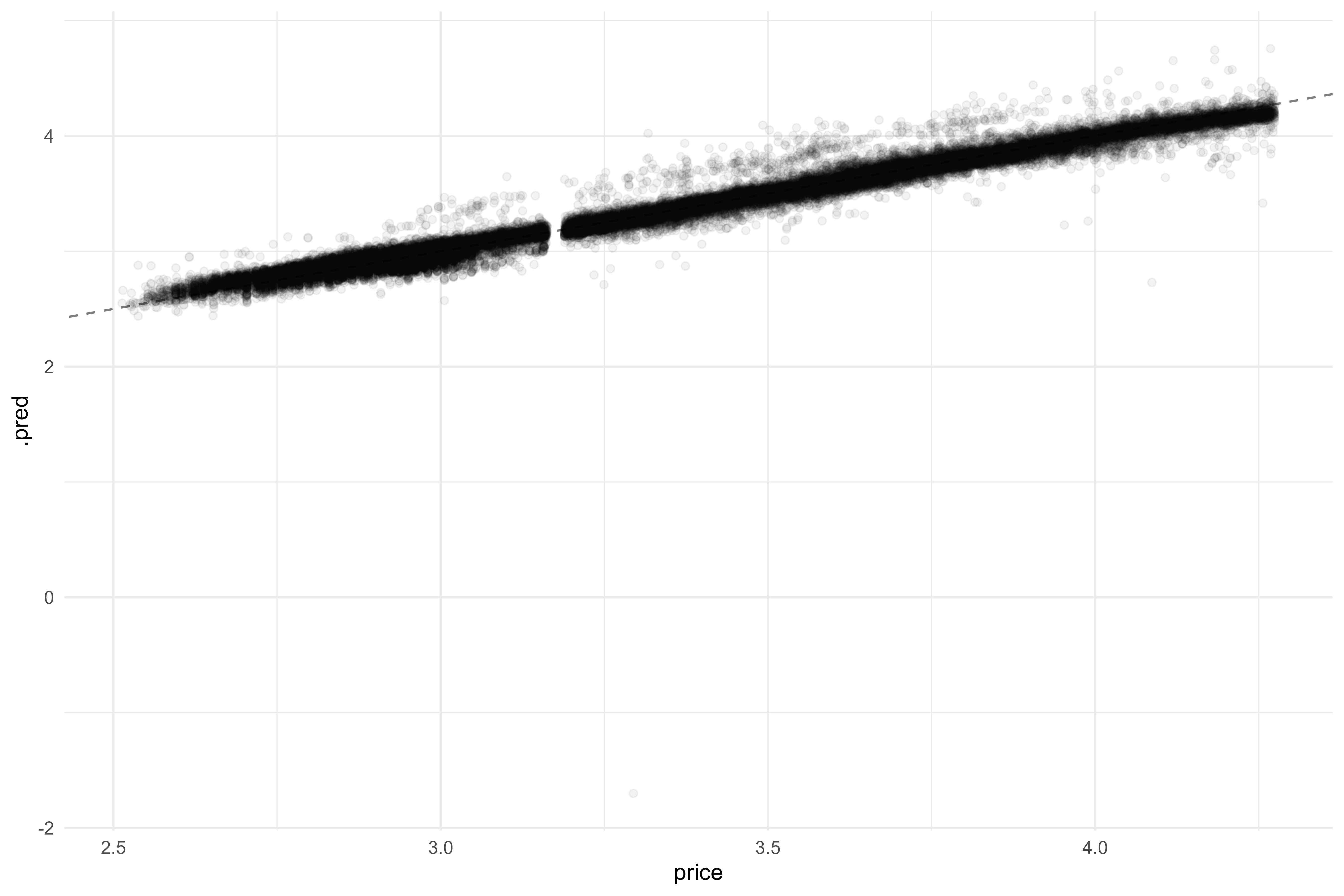That’s pretty good! We do have one value that’s way off, so let’s see if regulization can help. This will require setting a new baseline model, and we’ll tune our way to the best regularizaion parameters.

Code
``````metrics <-
rs07 %>%
collect_metrics() %>%
mutate(model = "model07") %>%
bind_rows(metrics)

rec08 <-
rec07 %>%
step_zv(all_numeric_predictors()) %>%
step_normalize(all_numeric_predictors(),
-color_E, -color_F, -color_G, -color_H, -color_I, -color_J,
-clarity_SI2, -clarity_VS1, -clarity_VS2, -clarity_VVS1, -clarity_VVS2, -clarity_other)

rm(mod05, mod06, mod07, rec05, rec06, rec07, wf05, wf06, wf07, rs05, rs06, rs07)

mod08 <-
linear_reg(penalty = tune(), mixture = tune()) %>%
set_engine("glmnet") %>%
set_mode("regression")

wf08 <-
workflow() %>%

diamonds_grid <-
grid_regular(penalty(), mixture(), levels = 20)

doParallel::registerDoParallel()
set.seed(5831)
rs08 <-
tune_grid(
wf08,
resamples = diamonds_folds,
control = ctrl_preds,
grid = diamonds_grid
)``````

Some notes but let’s explore our results…

Code
``````rs08 %>%
collect_metrics() %>%
ggplot(aes(x = penalty,
y = mean,
color = as.character(mixture))) +
geom_point() +
geom_line(alpha = 0.75) +
facet_wrap(~.metric, scales = "free") +
scale_x_log10()``````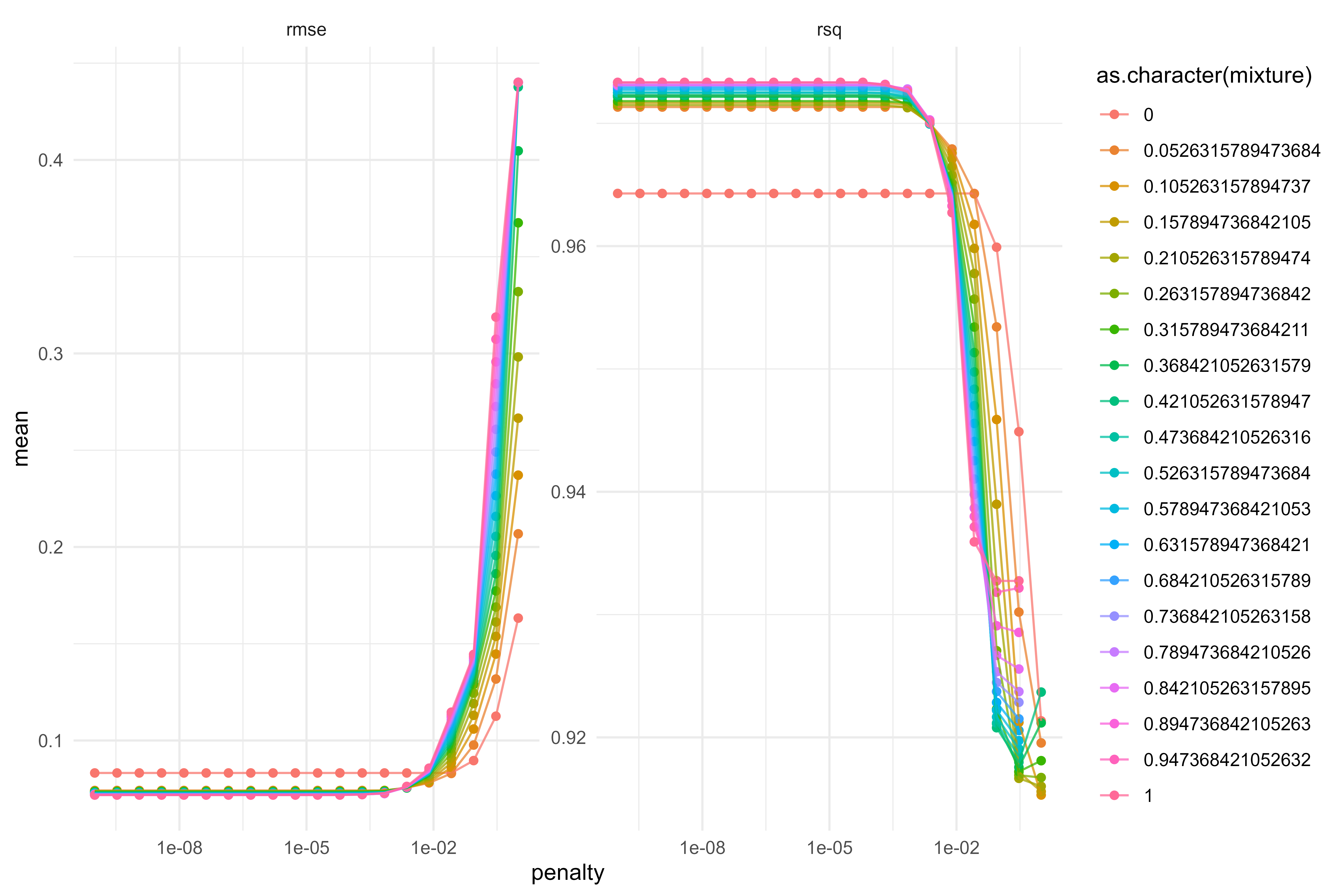Looks like we were performing pretty well with the unregularized model, oddly enough! Let’s select the best and finalize our workflow.

Code
``````best_metrics <-
rs08 %>%
select_best("rmse")

wf_final <-
finalize_workflow(wf08, best_metrics)

rm(mod08, rec07, rec08, rs08, wf08)

set.seed(333)
final_fit <-
wf_final %>%
fit(diamonds_train)

final_fit %>%
predict(diamonds_test) %>%
bind_cols(diamonds_test) %>%
select(price, .pred) %>%
ggplot(aes(x = price,
y = .pred)) +
geom_point(alpha = 0.05) +
geom_abline(alpha = 0.5,
linetype = "dashed",
size = 0.5)``````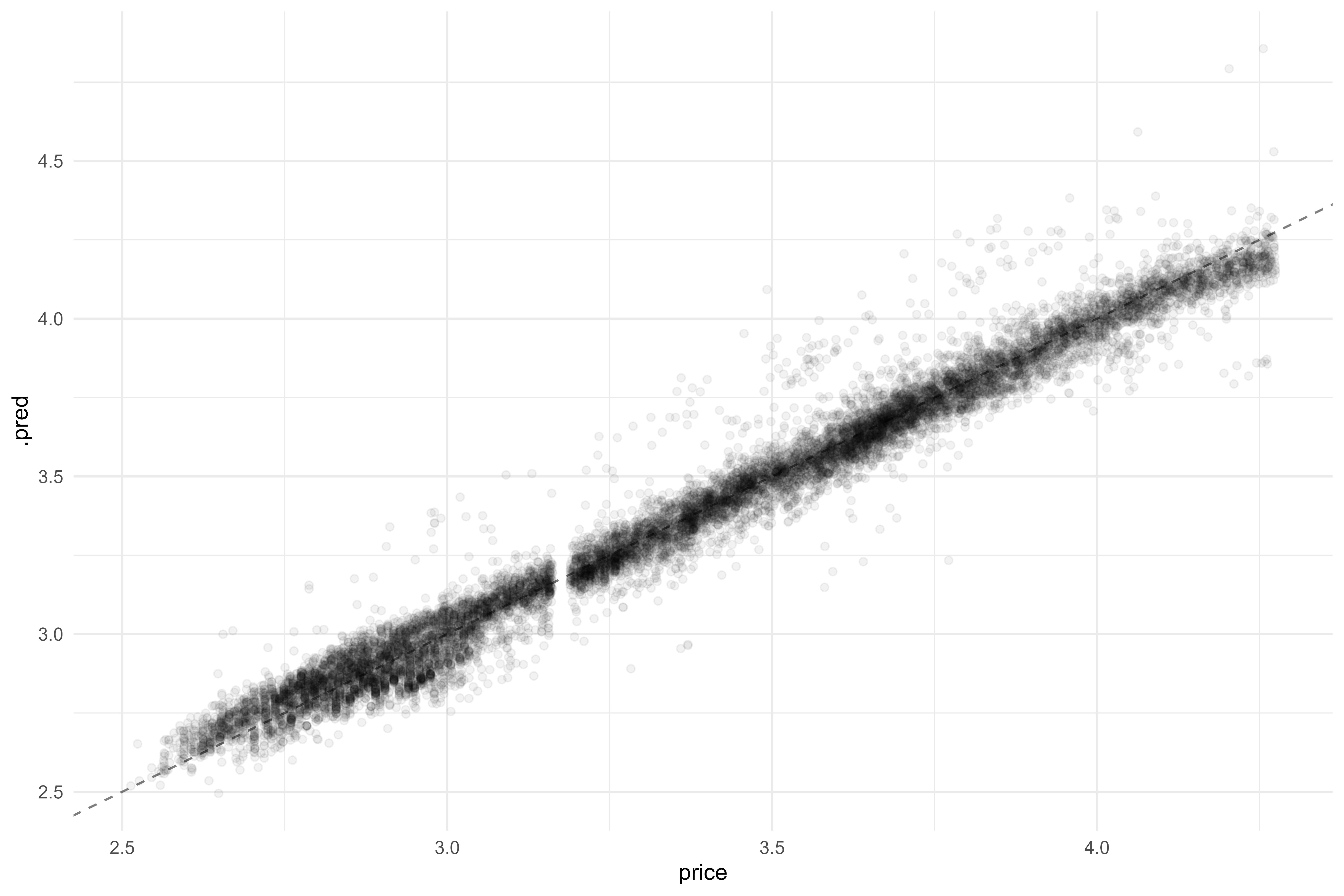What are the most important variables in this regularized model?

Code
``````final_fit %>%
pull_workflow_fit() %>%
vi(lambda = best_metrics\$penalty) %>%
mutate(Variable = fct_reorder(Variable, Importance)) %>%
ggplot(aes(x = Variable,
y = Importance,
fill = Sign)) +
geom_col() +
coord_flip()``````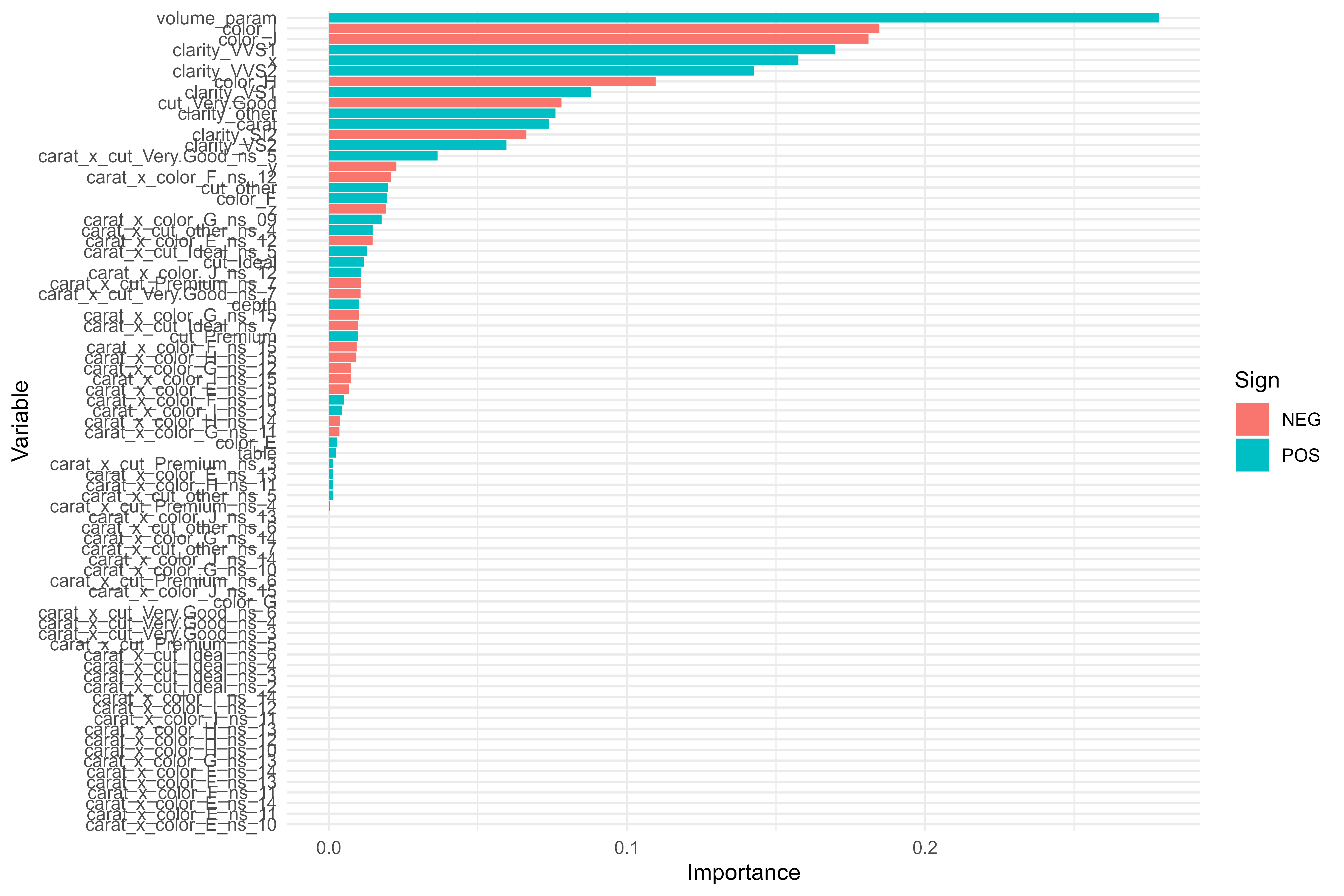As expected, most of our terms get regularized away, which is what we want! Our chart is a little unreadable; let’s plot just the most important variables in a few ways:

Code
``````final_fit %>%
pull_workflow_fit() %>%
vi(lambda = best_metrics\$penalty) %>%
arrange(desc(Importance)) %>%
mutate(Variable = fct_reorder(Variable, Importance)) %>%
ggplot(aes(x = Variable,
y = Importance,
fill = Sign)) +
geom_col() +
coord_flip()``````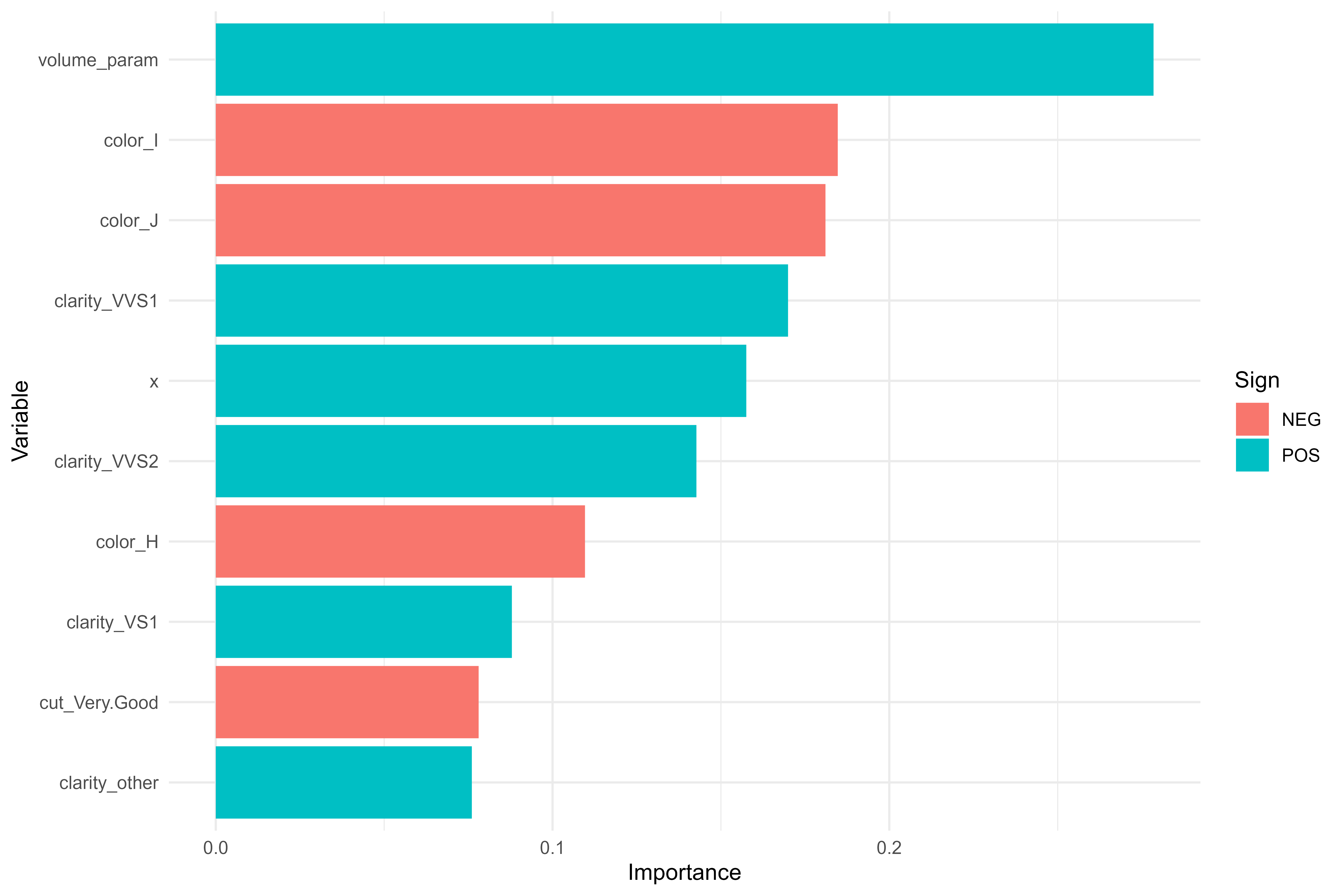Code
``````final_fit %>%
pull_workflow_fit() %>%
vi(lambda = best_metrics\$penalty) %>%
arrange(desc(Importance)) %>%
mutate(Importance = if_else(Sign == "NEG", -Importance, Importance),
Variable = fct_reorder(Variable, Importance)) %>%
ggplot(aes(x = Variable,
y = Importance,
fill = Sign)) +
geom_col() +
coord_flip()``````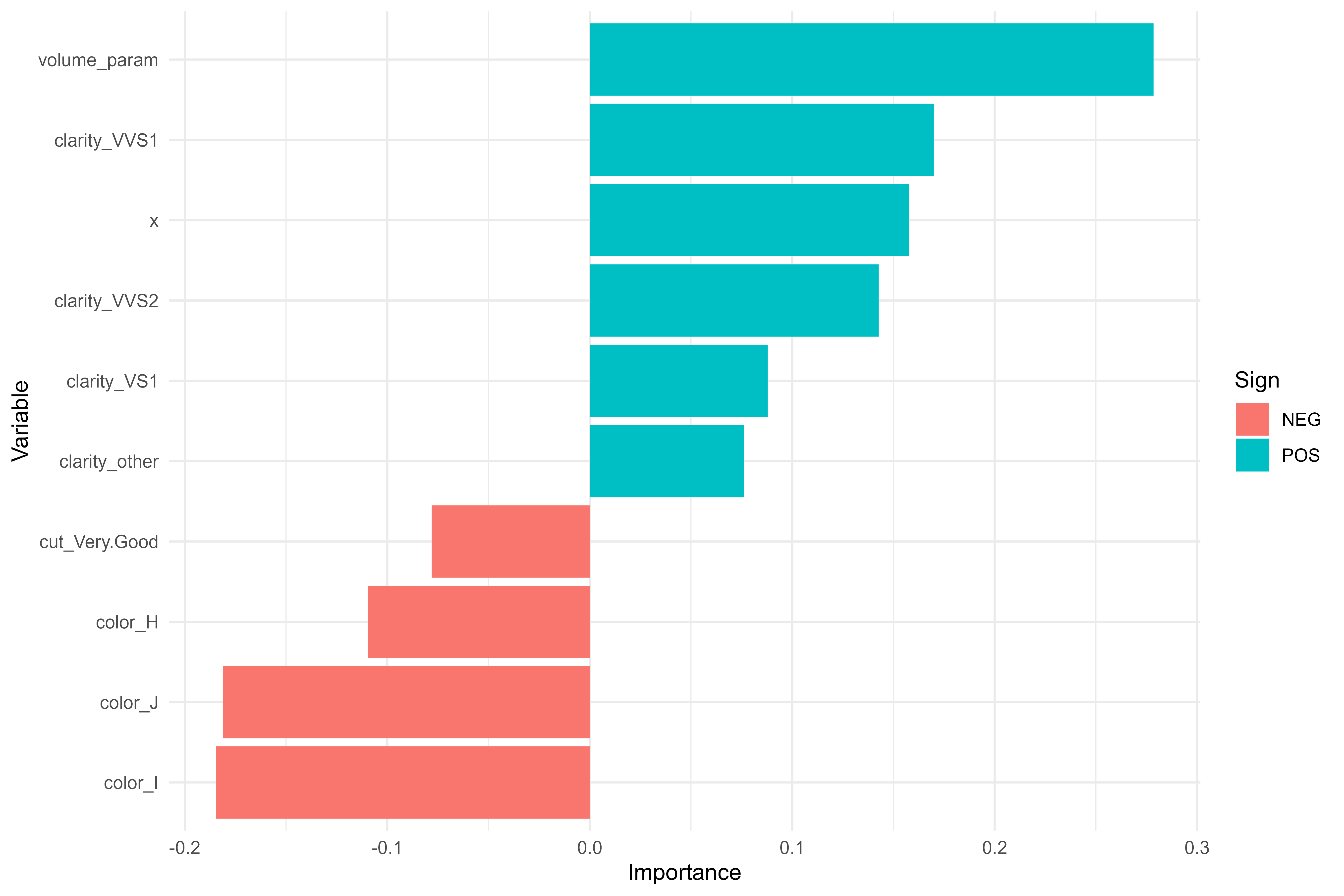And look at that! Our most important variable was one that came from feature engineering! The size of the rock had the biggest impact on price.

We’ve gone through a lot of steps, so it may be good to look back on what was done:

• Explored our dataset via some simple exploratory data analysis;
• Fit a simple linear model to predict the log-transform of price;
• Attempted (and failed) to improve upon the simple model with fancier normalization and resampling techniques;
• Explored the dataset further to find meaningful interactions and potential new features;
• Fit a new model with feature engineering;
• Tuned regularization parameters on our model with feature engineering to arrive at the final model.

Our models’ performances, ranked from best to worst, show that the final tuned model did indeed perform the best on the test dataset!

Code
``````final_preds <-
final_fit %>%
predict(diamonds_train) %>%
bind_cols(diamonds_train) %>%
select(price, .pred)

bind_rows(final_preds %>% rmse(price, .pred),
final_preds %>% rsq(price, .pred)) %>%
rename(mean = .estimate) %>%
select(-.estimator) %>%
mutate(model = "model_final") %>%
bind_rows(metrics %>% select(.metric, mean, model)) %>%
pivot_wider(names_from = .metric,
values_from = mean) %>%
mutate(model = fct_reorder(model, desc(rmse))) %>%
pivot_longer(rmse:rsq,
names_to = "metric",
values_to = "value") %>%
ggplot(aes(x = model,
y = value)) +
geom_point() +
facet_wrap(~metric, scales = "free") +
coord_flip()``````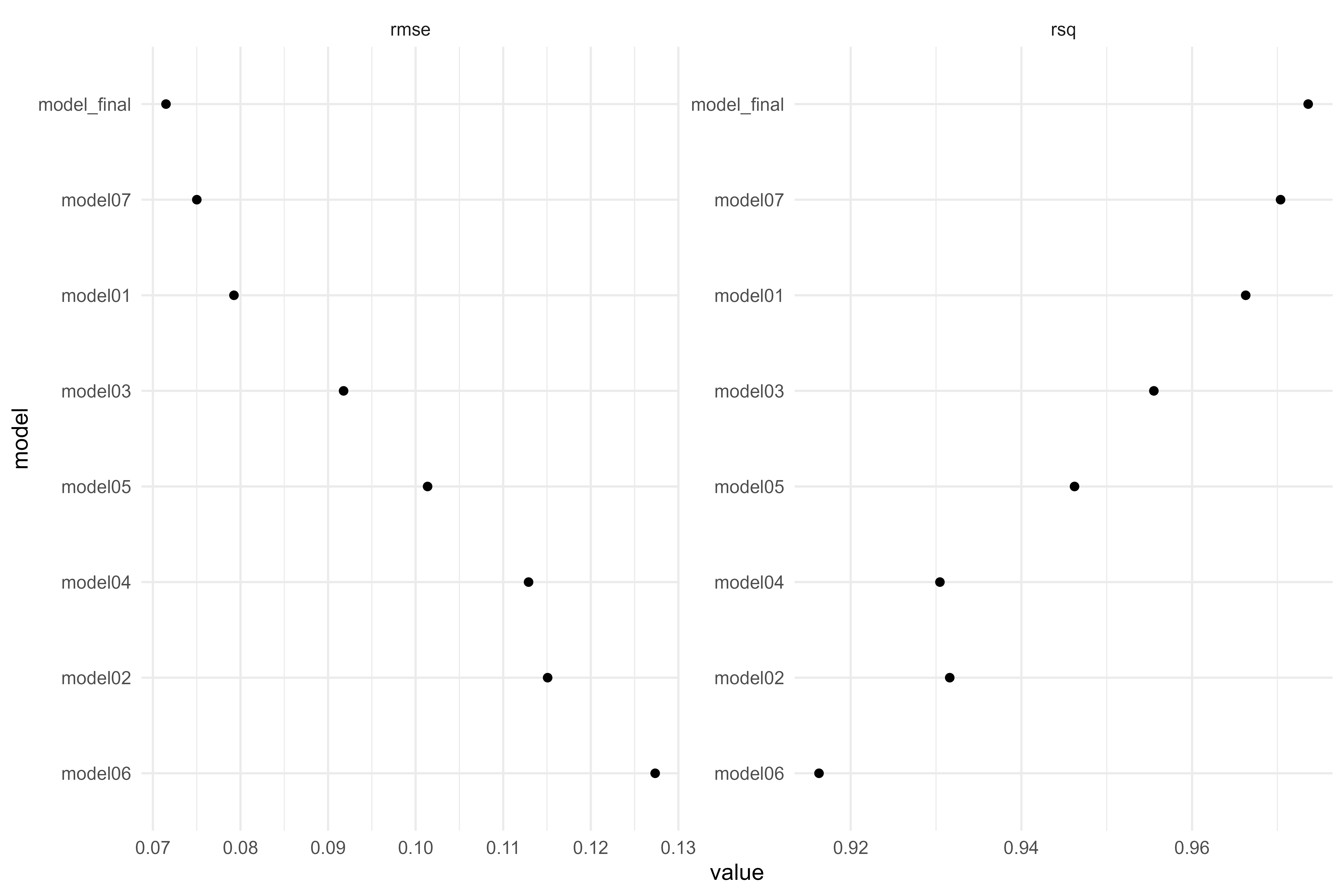## Citation

BibTeX citation:
``````@online{rieke2021,
author = {Rieke, Mark},
title = {Diamonds Are {Forever}},
date = {2021-11-14},